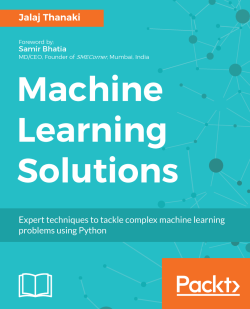# Machine Learning Solutions4 (1 reviews total)
By Jalaj Thanaki
• \$33.99 eBook
• \$41.99 Print + eBook
• \$12.99 eBook + Subscription
What do you get with a Packt Subscription?

• Constantly updated with 100+ new titles each month
• Breadth and depth in over 1,000+ technologies

Machine learning (ML) helps you find hidden insights from your data without the need for explicit programming. This book is your key to solving any kind of ML problem you might come across in your job.

You’ll encounter a set of simple to complex problems while building ML models, and you'll not only resolve these problems, but you’ll also learn how to build projects based on each problem, with a practical approach and easy-to-follow examples.

The book includes a wide range of applications: from analytics and NLP, to computer vision domains. Some of the applications you will be working on include stock price prediction, a recommendation engine, building a chat-bot, a facial expression recognition system, and many more. The problem examples we cover include identifying the right algorithm for your dataset and use cases, creating and labeling datasets, getting enough clean data to carry out processing, identifying outliers, overftting datasets, hyperparameter tuning, and more. Here, you'll also learn to make more timely and accurate predictions.

In addition, you'll deal with more advanced use cases, such as building a gaming bot, building an extractive summarization tool for medical documents, and you'll also tackle the problems faced while building an ML model. By the end of this book, you'll be able to fine-tune your models as per your needs to deliver maximum productivity.

Publication date:
April 2018
Publisher
Packt
Pages
566
ISBN
9781788390040

## Chapter 1. Credit Risk Modeling

All the chapters in this book are practical applications. We will develop one application per chapter. We will understand about the application, and choose the proper dataset in order to develop the application. After analyzing the dataset, we will build the base-line approach for the particular application. Later on, we will develop a revised approach that resolves the shortcomings of the baseline approach. Finally, we will see how we can develop the best possible solution using the appropriate optimization strategy for the given application. During this development process, we will learn necessary key concepts about Machine Learning techniques. I would recommend my reader run the code which is given in this book. That will help you understand concepts really well.

In this chapter, we will look at one of the many interesting applications of predictive analysis. I have selected the finance domain to begin with, and we are going to build an algorithm that can predict loan defaults. This is one of the most widely used predictive analysis applications in the finance domain. Here, we will look at how to develop an optimal solution for predicting loan defaults. We will cover all of the elements that will help us build this application.

We will cover the following topics in this chapter:

• Introducing the problem statement

• Understanding the dataset

• Understanding attributes of the dataset

• Data analysis

• Features engineering for the baseline model

• Selecting an ML algorithm

• Training the baseline model

• Understanding the testing matrix

• Testing the baseline model

• Problems with the existing approach

• How to optimize the existing approach

• Understanding key concepts to optimize the approach

• Hyperparameter tuning

• Implementing the revised approach

• Testing the revised approach

• Understanding the problem with the revised approach

• The best approach

• Implementing the best approach

• Summary

## Introducing the problem statement

First of all, let's try to understand the application that we want to develop or the problem that we are trying to solve. Once we understand the problem statement and it's use case, it will be much easier for us to develop the application. So let's begin!

Here, we want to help financial companies, such as banks, NBFS, lenders, and so on. We will make an algorithm that can predict to whom financial institutes should give loans or credit. Now you may ask what is the significance of this algorithm? Let me explain that in detail. When a financial institute lends money to a customer, they are taking some kind of risk. So, before lending, financial institutes check whether or not the borrower will have enough money in the future to pay back their loan. Based on the customer's current income and expenditure, many financial institutes perform some kind of analysis that helps them decide whether the borrower will be a good customer for that bank or not. This kind of analysis is manual and time-consuming. So, it needs some kind of automation. If we develop an algorithm, that will help financial institutes gauge their customers efficiently and effectively.Your next question may be what is the output of our algorithm? Our algorithm will generate probability. This probability value will indicate the chances of borrowers defaulting. Defaulting means borrowers cannot repay their loan in a certain amount of time. Here, probability indicates the chances of a customer not paying their loan EMI on time, resulting in default. So, a higher probability value indicates that the customer would be a bad or inappropriate borrower (customer) for the financial institution, as they may default in the next 2 years. A lower probability value indicates that the customer will be a good or appropriate borrower (customer) for the financial institution and will not default in the next 2 years.

Here, I have given you information regarding the problem statement and its output, but there is an important aspect of this algorithm: its input. So, let's discuss what our input will be!

## Understanding the dataset

Here, we are going to discuss our input dataset in order to develop the application. You can find the dataset at https://github.com/jalajthanaki/credit-risk-modelling/tree/master/data.

Let's discuss the dataset and its attributes in detail. Here, in the dataset, you can find the following files:

• `cs-training.csv`

• Records in this file are used for training, so this is our training dataset.

• `cs-test.csv`

• Records in this file are used for testing our machine learning models, so this is our testing dataset.

• `Data Dictionary.xls`

• This file contains information about each of the attributes of the dataset. So, this file is referred to as our data dictionary.

• `sampleEntry.csv`

• This file gives us an idea about the format in which we need to generate our end output for our testing dataset. If you open this file, then you will see that we need to generate the probability of each of the records present in the testing dataset. This probability value indicates the chances of borrowers defaulting.

### Understanding attributes of the dataset

The dataset has 11 attributes, which are shown as follows: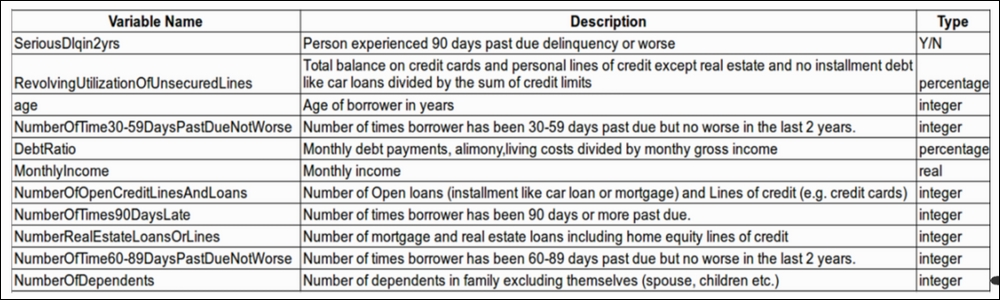Figure 1.1: Attributes (variables) of the dataset

We will look at each of the attributes one by one and understand their meaning in the context of the application:

1. SeriousDlqin2yrs:

• In the dataset, this particular attribute indicates whether the borrower has experienced any past dues until 90 days in the previous 2 years.

• The value of this attribute is Yes if the borrower has experienced past dues of more than 90 days in the previous 2 years. If the EMI was not paid by the borrower 90 days after the due date of the EMI, then this flag value is Yes.

• The value of this attribute is No if the borrower has not experienced past dues of more than 90 days in the previous 2 years. If the EMI was paid by the borrower before 90 days from the due date of the EMI, then this flag value is No.

• This attribute has target labels. In other words, we are going to predict this value using our algorithm for the test dataset.

2. RevolvingUtilizationOfUnsecuredLines:

• This attribute indicates the credit card limits of the borrower after excluding any current loan debt and real estate.

• Suppose I have a credit card and its credit limit is \$1,000. In my personal bank account, I have \$1,000. My credit card balance is \$500 out of \$1,000.

• So, the total maximum balance I can have via my credit card and personal bank account is \$1,000 + \$1,000 = \$2,000; I have used \$500 from my credit card limit, so the total balance that I have is \$500 (credit card balance) + \$1,000 (personal bank account balance) = \$1,500.

• If account holder have taken home loan or other property loan and paying EMIs for those loan then we are not considering EMI value for property loan. Here, for this data attribute we have considered account holder's credit card balance and personal account balance.

• So, the RevolvingUtilizationOfUnsecuredLines value is = \$1,500 / \$2,000 = 0.7500

3. Age:

• This attribute is self-explanatory. It indicates the borrower's age.

4. NumberOfTime30-59DaysPastDueNotWorse:

• The number of this attribute indicates the number of times borrowers have paid their EMIs late but have paid them 30 days after the due date or 59 days before the due date.

5. DebtRatio:

• This is also a self-explanatory attribute, but we will try and understand it better with an example.

• If my monthly debt is \$200 and my other expenditure is \$500, then I spend \$700 monthly. If my monthly income is \$1,000, then the value of the DebtRatio is \$700/\$1,000 = 0.7000

6. MonthlyIncome:

• This attribute contains the value of the monthly income of borrowers.

7. NumberOfOpenCreditLinesAndLoans:

• This attribute indicates the number of open loans and/or the number of credit cards the borrower holds.

8. NumberOfTimes90DaysLate:

• This attribute indicates how many times a borrower has paid their dues 90 days after the due date of their EMIs.

9. NumberRealEstateLoansOrLines:

• This attribute indicates the number of loans the borrower holds for their real estate or the number of home loans a borrower has.

10. NumberOfTime60-89DaysPastDueNotWorse:

• This attribute indicates how many times borrowers have paid their EMIs late but paid them 60 days after their due date or 89 days before their due date.

11. NumberOfDependents:

• This attribute is self-explanatory as well. It indicates the number of dependent family members the borrowers have. The dependent count is excluding the borrower.

These are basic attribute descriptions of the dataset, so you have a basic idea of the kind of dataset we have. Now it's time to get hands-on. So from the next section onward, we will start coding. We will begin exploring our dataset by performing basic data analysis so that we can find out the statistical properties of the dataset.

### Data analysis

This section is divided into two major parts. You can refer to the following figure to see how we will approach this section: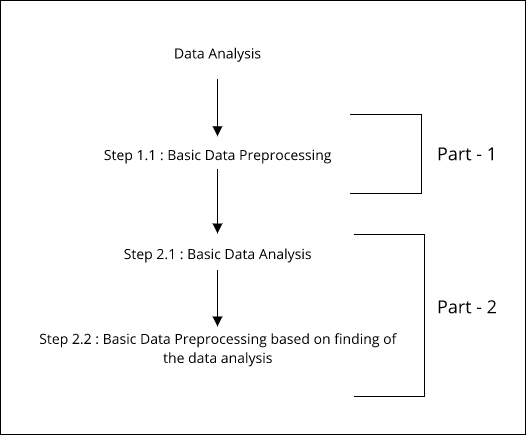Figure 1.2: Parts and steps of data analysis

In the first part, we have only one step. In the preceding figure, this is referred to as step 1.1. In this first step, we will do basic data preprocessing. Once we are done with that, we will start with our next part.

The second part has two steps. In the figure, this is referred to as step 2.1. In this step, we will perform basic data analysis using statistical and visualization techniques, which will help us understand the data. By doing this activity, we will get to know some statistical facts about our dataset. After this, we will jump to the next step, which is referred to as step 2.2 in Figure 1.2. In this step, we will once again perform data preprocessing, but, this time, our preprocessing will be heavily based on the findings that we have derived after doing basic data analysis on the given training dataset. You can find the code at this GitHub Link: https://github.com/jalajthanaki/credit-risk-modelling/blob/master/basic_data_analysis.ipynb.

So let's begin!

#### Data preprocessing

In this section, we will perform a minimal amount of basic preprocessing. We will look at the approaches as well as their implementation.

##### First change

If you open the `cs-training.csv` file, then you will find that there is a column without a heading, so we will add a heading there. Our heading for that attribute is `ID`. If you want to drop this column, you can because it just contains the `sr.no` of the records.

##### Second change

This change is not a mandatory one. If you want to skip it, you can, but I personally like to perform this kind of preprocessing. The change is related to the heading of the attributes, we are removing "-" from the headers. Apart from this, I will convert all the column heading into lowercase. For example, the attribute named `NumberOfTime60-89DaysPastDueNotWorse` will be converted into `numberoftime6089dayspastduenotworse`. These kinds of changes will help us when we perform in-depth data analysis. We do not need to take care of this hyphen symbols while processing.

##### Implementing the changes

Now, you may ask how will I perform the changes described? Well, there are two ways. One is a manual approach. In this approach, you will open the `cs-training.csv` file and perform the changes manually. This approach certainly isn't great. So, we will take the second approach. With the second approach, we will perform the changes using Python code. You can find all the changes in the following code snippets.

Refer to the following screenshot for the code to perform the first change: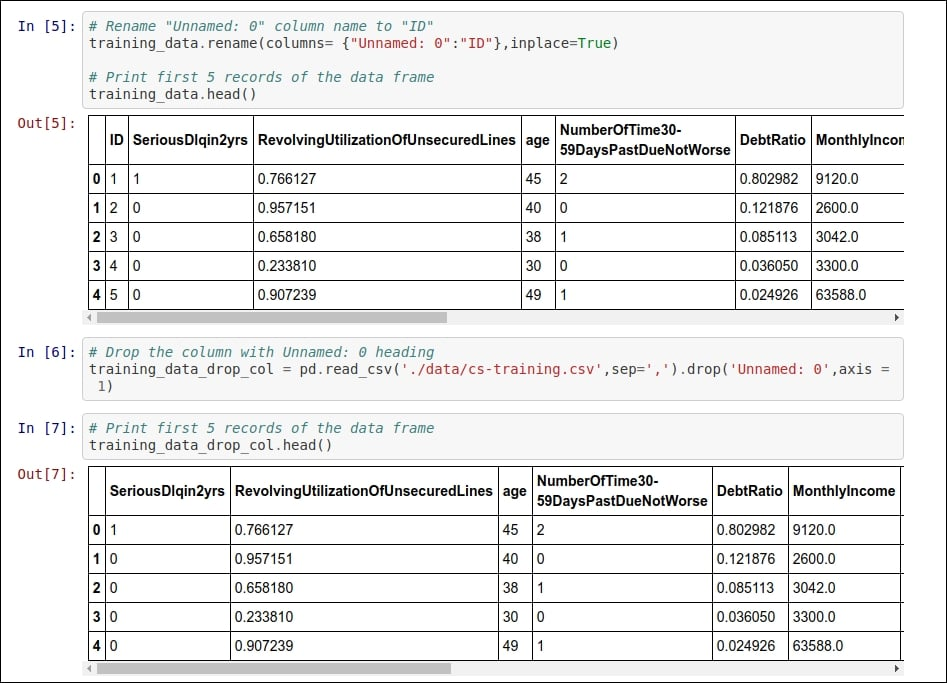Figure 1.3: Code snippet for implementing the renaming or dropping of the index column

For the second change, you can refer to Figure 1.4: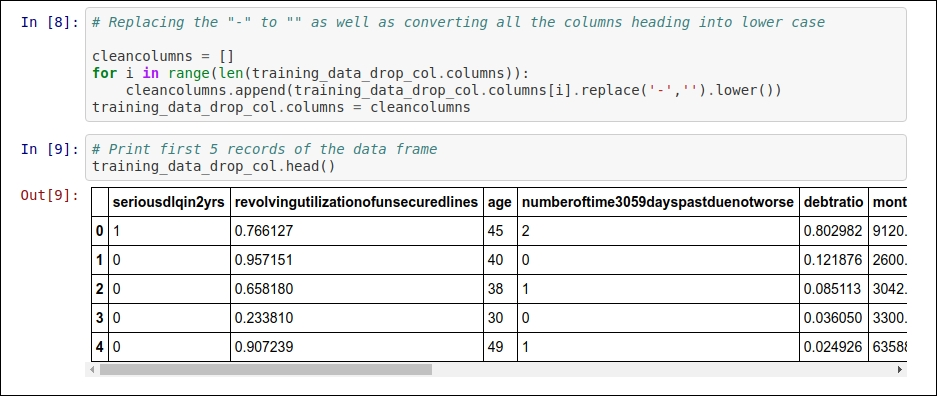Figure 1.4: Code snippet for removing "-" from the column heading and converting all the column headings into lowercase

The same kind of preprocessing needs to be done on the `cs-test.csv` file. This is because the given changes are common for both the training and testing datasets.

You can find the entire code on GitHub by clicking on this link: https://github.com/jalajthanaki/credit-risk-modelling/blob/master/basic_data_analysis.ipynb.

You can also move hands-on along with reading.

I'm using Python 2.7 as well as a bunch of different Python libraries for the implementation of this code. You can find information related to Python dependencies as well as installation in the README section. Now let's move on to the basic data analysis section.

#### Basic data analysis followed by data preprocessing

Let's perform some basic data analysis, which will help us find the statistical properties of the training dataset. This kind of analysis is also called exploratory data analysis (EDA), and it will help us understand how our dataset represents the facts. After deriving some facts, we can use them in order to derive feature engineering. So let's explore some important facts!

From this section onward, all the code is part of one iPython notebook. You can refer to the code using this GitHub Link: https://github.com/jalajthanaki/credit-risk-modelling/blob/master/Credit%20Risk%20Analysis.ipynb.

The following are the steps we are going to perform:

1. Listing statistical properties

2. Finding the missing values

3. Replacing missing values

4. Correlation

5. Detecting Outliers

##### Listing statistical properties

In this section, we will get an idea about the statistical properties of the training dataset. Using pandas' describe function, we can find out the following basic things:

• `count`: This will give us an idea about the number of records in our training dataset.

• `mean`: This value gives us an indication of the mean of each of the data attributes.

• `std`: This value indicates the standard deviation for each of the data attributes. You can refer to this example: http://www.mathsisfun.com/data/standard-deviation.html.

• `min`: This value gives us an idea of what the minimum value for each of the data attributes is.

• `25%`: This value indicates the 25th percentile. It should fall between 0 and 1.

• `50%`: This value indicates the 50th percentile. It should fall between 0 and 1.

• `75%`: This value indicates the 75th percentile. It should fall between 0 and 1.

• `max`: This value gives us an idea of what the maximum value for each of the data attributes is.

Take a look at the code snippet in the following figure: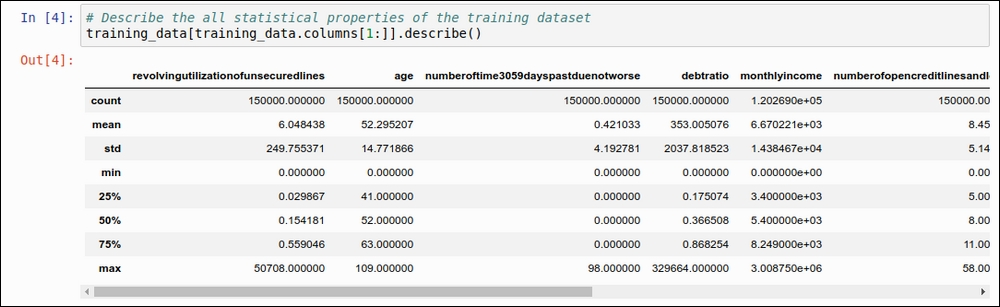Figure 1.5: Basic statistical properties using the describe function of pandas

We need to find some other statistical properties for our dataset that will help us understand it. So, here, we are going to find the median and mean for each of the data attributes. You can see the code for finding the median in the following figure: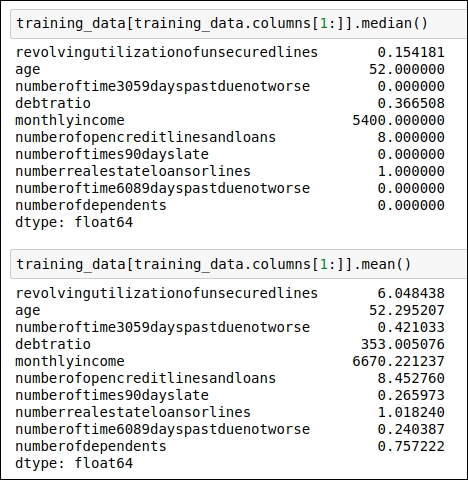Figure 1.6: Code snippet for generating the median and the mean for each data attribute

Now let's check out what kind of data distribution is present in our dataset. We draw the frequency distribution for our target attribute, `seriousdlqin2yrs`, in order to understand the overall distribution of the target variable for the training dataset. Here, we will use the `seaborn` visualization library. You can refer to the following code snippet: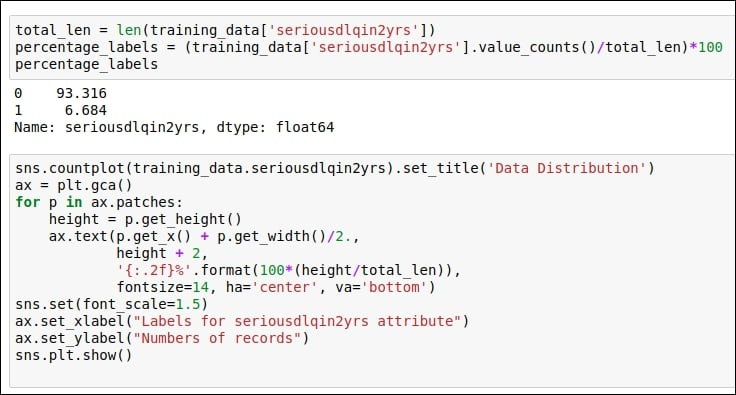Figure 1.7: Code snippet for understanding the target variable distribution as well as the code snippet for the visualization of the distribution

You can refer to the visualization chart in the following figure: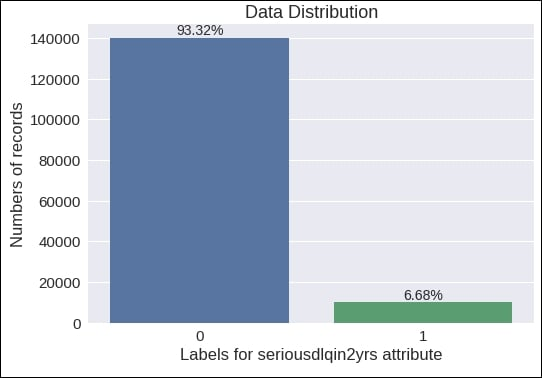Figure 1.8: Visualization of the variable distribution of the target data attribute

From this chart, you can see that there are many records with the target label 0 and fewer records with the target label 1. You can see that the data records with a 0 label are about 93.32%, whereas 6.68% of the data records are labeled 1. We will use all of these facts in the upcoming sections. For now, we can consider our outcome variable as imbalanced.

##### Finding missing values

In order to find the missing values in the dataset, we need to check each and every data attribute. First, we will try to identify which attribute has a missing or null value. Once we have found out the name of the data attribute, we will replace the missing value with a more meaningful value. There are a couple of options available for replacing the missing values. We will explore all of these possibilities.

Let's code for our first step. Here, we will see which data attribute has missing values as well count how many records there are for each data attribute with a missing value. You can see the code snippet in the following figure: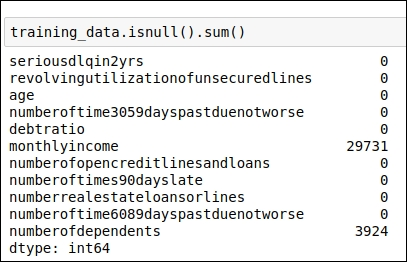Figure 1.9: Code snippet for identifying which data attributes have missing values

As displayed in the preceding figure, the following two data attributes have missing values:

• `monthlyincome`: This attribute contains 29,731 records with a missing value.

• `numberofdependents`: This attribute contains 3,924 records with a missing value.

You can also refer to the code snippet in the following figure for the graphical representation of the facts described so far: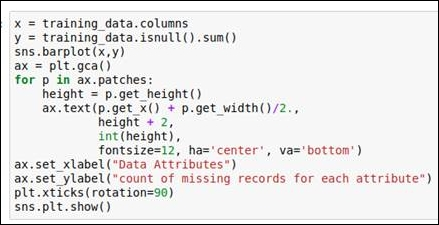Figure 1.10: Code snippet for generating a graph of missing values

You can view the graph itself in the following figure: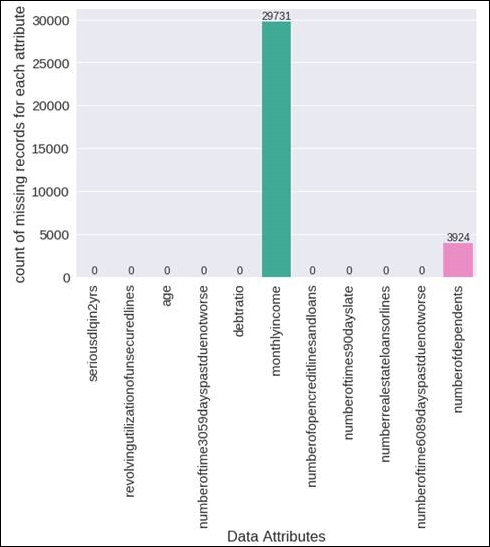Figure 1.11: A graphical representation of the missing values

In this case, we need to replace these missing values with more meaningful values. There are various standard techniques that we can use for that. We have the following two options:

• Replace the missing value with the mean value of that particular data attribute

• Replace the missing value with the median value of that particular data attribute

In the previous section, we already derived the mean and median values for all of our data attributes, and we will use them. Here, our focus will be on the attributes titled `monthlyincome` and `numberofdependents` because they have missing values. We have found out which data attributes have missing values, so now it's time to perform the actual replacement operation. In the next section, you will see how we can replace the missing values with the mean or the median.

##### Replacing missing values

In the previous section, we figured out which data attributes in our training dataset contain missing values. We need to replace the missing values with either the mean or the median value of that particular data attribute. So in this section, we will focus particularly on how we can perform the actual replacement operation. This operation of replacing the missing value is also called imputing the missing data.

Before moving on to the code section, I feel you guys might have questions such as these: should I replace missing values with the mean or the median? Are there any other options available? Let me answer these questions one by one.

The answer to the first question, practically, will be a trial and error method. So you first replace missing values with the mean value, and during the training of the model, measure whether you get a good result on the training dataset or not. Then, in the second iteration, we need to try to replace the values with the median and measure whether you get a good result on the training dataset or not.

In order to answer the second question, there are many different imputation techniques available, such as the deletion of records, replacing the values using the KNN method, replacing the values using the most frequent value, and so on. You can select any of these techniques, but you need to train the model and measure the result. Without implementing a technique, you can't really say with certainty that a particular imputation technique will work for the given training dataset. Here, we are talking in terms of the credit-risk domain, so I would not get into the theory much, but just to refresh your concepts, you can refer to the following articles:

We can see the code for replacing the missing values using the attribute's mean value and its median value in the following figure: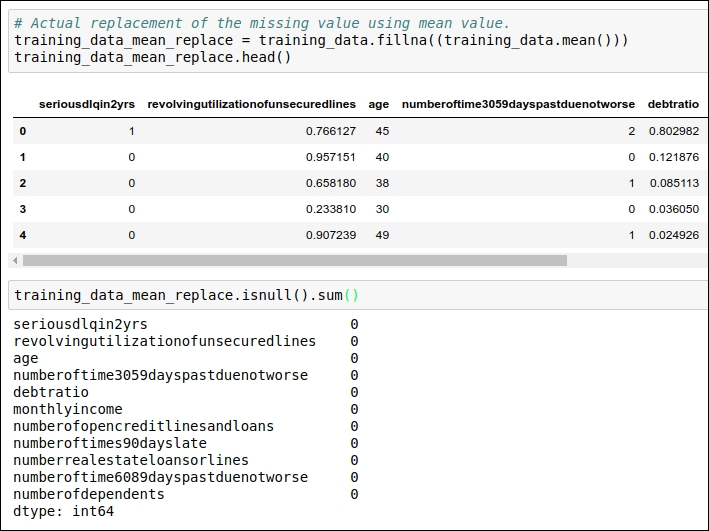Figure 1.12: Code snippet for replacing the mean values

In the preceding code snippet, we replaced the missing value with the mean value, and in the second step, we verified that all the missing values have been replaced with the mean of that particular data attribute.

In the next code snippet, you can see the code that we have used for replacing the missing values with the median of those data attributes. Refer to the following figure: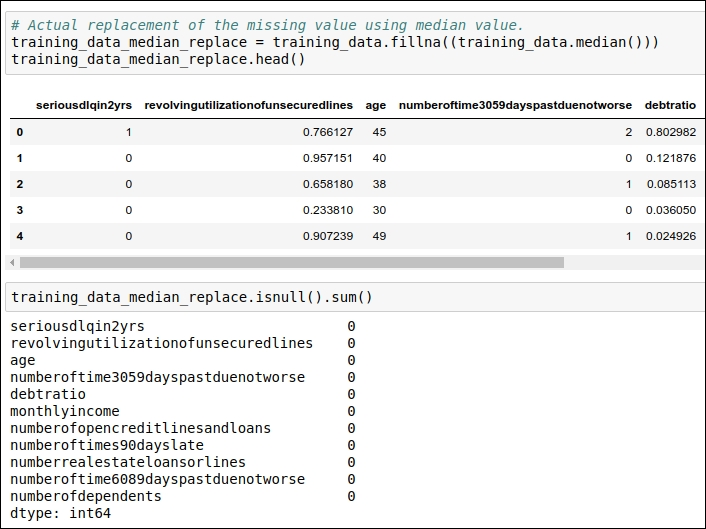Figure 1.13: Code snippet for replacing missing values with the median

In the preceding code snippet, we have replaced the missing value with the median value, and in second step, we have verified that all the missing values have been replaced with the median of that particular data attribute.

In the first iteration, I would like to replace the missing value with the median.

In the next section, we will see one of the important aspects of basic data analysis: finding correlations between data attributes. So, let's get started with correlation.

##### Correlation

I hope you basically know what correlation indicates in machine learning. The term correlation refers to a mutual relationship or association between quantities. If you want to refresh the concept on this front, you can refer to https://www.investopedia.com/terms/c/correlation.asp.

So, here, we will find out what kind of association is present among the different data attributes. Some attributes are highly dependent on one or many other attributes. Sometimes, values of a particular attribute increase with respect to its dependent attribute, whereas sometimes values of a particular attribute decrease with respect to its dependent attribute. So, correlation indicates the positive as well as negative associations among data attributes. You can refer to the following code snippet for the correlation: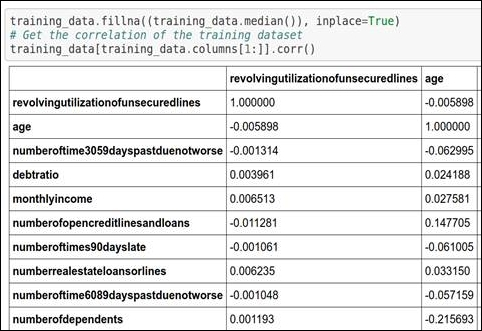Figure 1.14: Code snippet for generating correlation

You can see the code snippet of the graphical representation of the correlation in the following figure: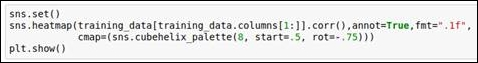Figure 1.15: Code snippet for generating a graphical snippet

You can see the graph of the correlation in the following figure: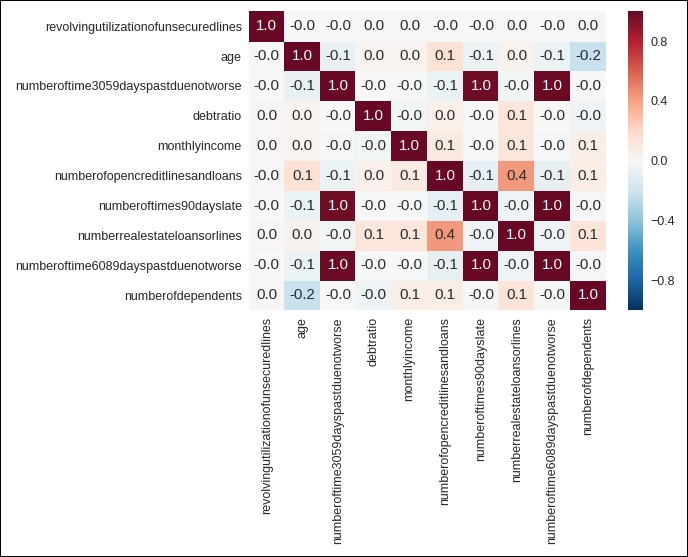Figure 1.16: Heat map for correlation

Let's look at the preceding graph because it will help you understand correlation in a great way. The following facts can be derived from the graph:

• Cells with 1.0 values are highly associated with each other.

• Each attribute has a very high correlation with itself, so all the diagonal values are 1.0.

• The data attribute numberoftime3059dayspastduenotworse (refer to the data attribute given on the vertical line or on the y axis) is highly associated with two attributes, numberoftimes90dayslate and numberoftime6089dayspastduenotworse. These two data attributes are given on the x axis (or on the horizontal line).

• The data attribute numberoftimes90dayslate is highly associated with numberoftime3059dayspastduenotworse and numberoftime6089dayspastduenotworse. These two data attributes are given on the x axis (or on the horizontal line).

• The data attribute numberoftime6089dayspastduenotworse is highly associated with numberoftime3059dayspastduenotworse and numberoftimes90dayslate. These two data attributes are given on the x axis (or on the horizontal line).

• The data attribute numberofopencreditlinesandloans also has an association with numberrealestateloansorlines and vice versa. Here, the data attribute numberrealestateloansorlines is present on the x axis (or on the horizontal line).

Before moving ahead, we need to check whether these attributes contain any outliers or insignificant values. If they do, we need to handle these outliers, so our next section is about detecting outliers from our training dataset.

##### Detecting outliers

In this section, you will learn how to detect outliers as well as how to handle them. There are two steps involved in this section:

• Outliers detection techniques

• Handling outliers

First, let's begin with detecting outliers. Now you guys might have wonder why should we detect outliers. In order to answer this question, I would like to give you an example. Suppose you have the weights of 5-year-old children. You measure the weight of five children and you want to find out the average weight. The children weigh 15, 12, 13, 10, and 35 kg. Now if you try to find out the average of these values, you will see that the answer 17 kg. If you look at the weight range carefully, then you will realize that the last observation is out of the normal range compared to the other observations. Now let's remove the last observation (which has a value of 35) and recalculate the average of the other observations. The new average is 12.5 kg. This new value is much more meaningful in comparison to the last average value. So, the outlier values impact the accuracy greatly; hence, it is important to detect them. Once that is done, we will explore techniques to handle them in upcoming section named handling outlier.

##### Outliers detection techniques

Here, we are using the following outlier detection techniques:

• Percentile-based outlier detection

• Median Absolute Deviation (MAD)-based outlier detection

• Standard Deviation (STD)-based outlier detection

• Majority-vote-based outlier detection

• Visualization of outliers

##### Percentile-based outlier detection

Here, we have used percentile-based outlier detection, which is derived based on the basic statistical understanding. We assume that we should consider all the data points that lie under the percentile range from 2.5 to 97.5. We have derived the percentile range by deciding on a threshold of 95. You can refer to the following code snippet: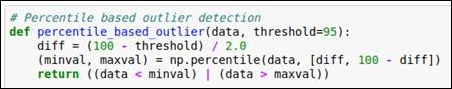Figure 1.17: Code snippet for percentile-based outlier detection

We will use this method for each of the data attributes and detect the outliers.

##### Median Absolute Deviation (MAD)-based outlier detection

MAD is a really simple statistical concept. There are four steps involved in it. This is also known as modified Z-score. The steps are as follows:

1. Find the median of the particular data attribute.

2. For each of the given values for the data attribute, subtract the previously found median value. This subtraction is in the form of the absolute value. So, for each data point, you will get the absolute value.

3. In the third step, generate the median of the absolute values that we derived in the second step. We will perform this operation for each data point for each of the data attributes. This value is called the MAD value.

4. In the fourth step, we will use the following equation to derive the modified Z-score: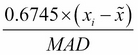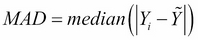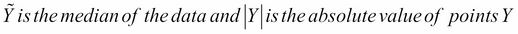Now it's time to refer to the following code snippet: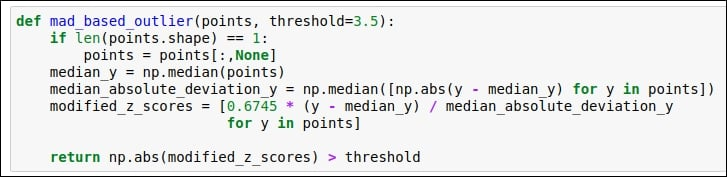Figure 1.18: Code snippet for MAD-based outlier detection

##### Standard Deviation (STD)-based outlier detection

In this section, we will use standard deviation and the mean value to find the outlier. Here, we select a random threshold value of 3. You can refer to the following code snippet: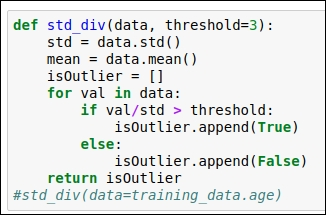Figure 1.19: Standard Deviation (STD) based outlier detection code

##### Majority-vote-based outlier detection:

In this section, we will build the voting mechanism so that we can simultaneously run all the previously defined methods—such as percentile-based outlier detection, MAD-based outlier detection, and STD-based outlier detection—and get to know whether the data point should be considered an outlier or not. We have seen three techniques so far. So, if two techniques indicate that the data should be considered an outlier, then we consider that data point as an outlier; otherwise, we don't. So, the minimum number of votes we need here is two. Refer to the following figure for the code snippet: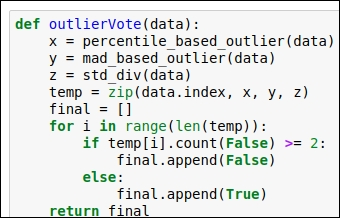Figure 1.20: Code snippet for the voting mechanism for outlier detection

##### Visualization of outliers

In this section, we will plot the data attributes to get to know about the outliers visually. Again, we are using the `seaborn` and `matplotlib` library to visualize the outliers. You can find the code snippet in the following figure: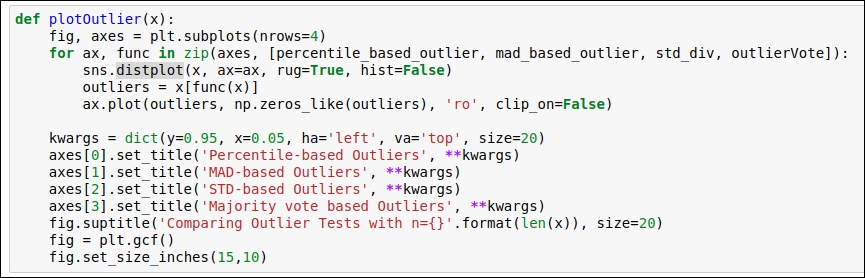Figure 1.21: Code snippet for the visualization of the outliers

Refer to the preceding figure for the graph and learn how our defined methods detect the outlier. Here, we chose a sample size of 5,000. This sample was selected randomly.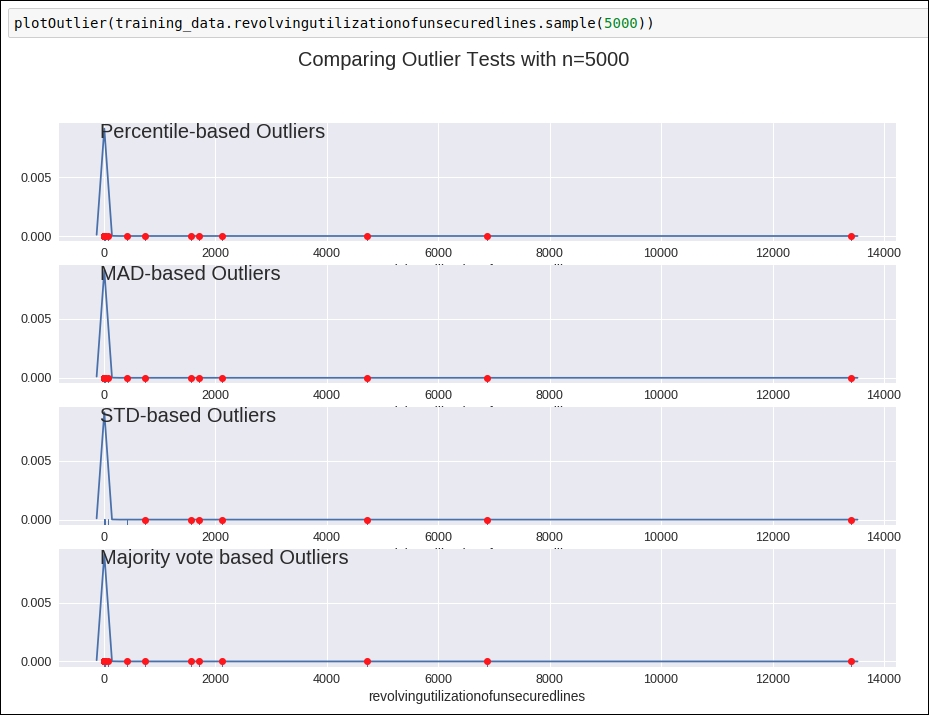Figure 1.22: Graph for outlier detection

Here, you can see how all the defined techniques will help us detect outlier data points from a particular data attribute. You can see all the attribute visualization graphs on this GitHub link at https://github.com/jalajthanaki/credit-risk-modelling/blob/master/Credit%20Risk%20Analysis.ipynb.

So far, you have learned how to detect outliers, but now it's time to handle these outlier points. In the next section, we will look at how we can handle outliers.

##### Handling outliers

In this section, you will learn how to remove or replace outlier data points. This particular step is important because if you just identify the outlier but aren't able to handle it properly, then at the time of training, there will be a high chance that we over-fit the model. So, let's learn how to handle the outliers for this dataset. Here, I will explain the operation by looking at the data attributes one by one.

##### Revolving utilization of unsecured lines

In this data attribute, when you plot an outlier detection graph, you will come to know that values of more than 0.99999 are considered outliers. So, values greater than 0.99999 can be replaced with 0.99999. So for this data attribute, we perform the replacement operation. We have generated new values for the data attribute `revolvingutilizationofunsecuredlines`.

For the code, you can refer to the following figure: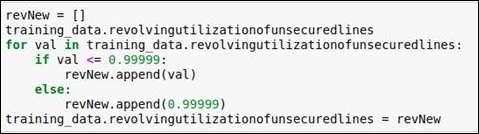Figure 1.23: Code snippet for replacing outlier values with 0.99999

##### Age

In this attribute, if you explore the data and see the percentile-based outlier, then you see that there is an outlier with a value of 0 and the youngest age present in the data attribute is 21. So, we replace the value of 0 with 22. We code the condition such that the age should be more than 22. If it is not, then we will replace the age with 22. You can refer to the following code and graph.

The following figure shows how the frequency distribution of age is given in the dataset. By looking at the data, we can derive the fact that 0 is the outlier value: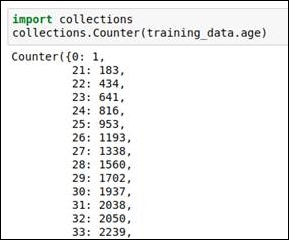Figure 1.24: Frequency for each data value shows that 0 is an outlier

Refer to the following box graph, which gives us the distribution indication of the age: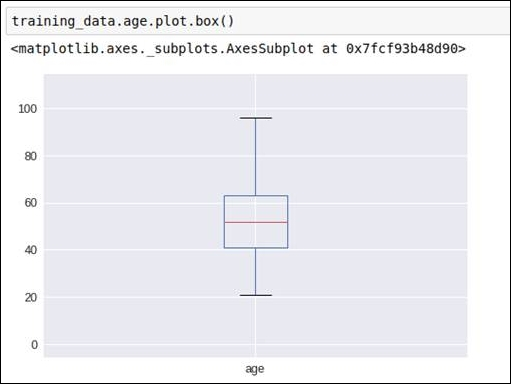Figure 1.25: Box graph for the age data attribute

Before removing the outlier, we got the following outlier detection graph: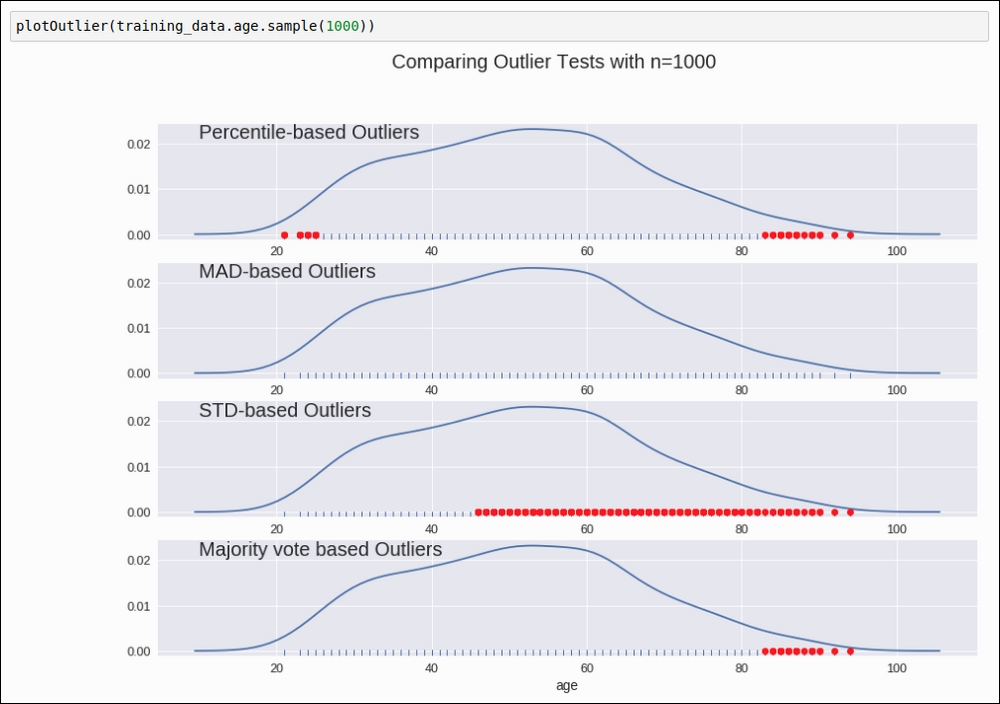Figure 1.26: Graphical representation of detecting outliers for data attribute age

The code for replacing the outlier is as follows: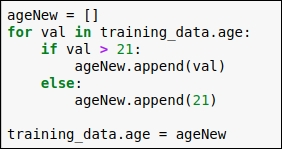Figure 1.27: Replace the outlier with the minimum age value 21

In the code, you can see that we have checked each data point of the age column, and if the age is greater than 21, then we haven't applied any changes, but if the age is less than 21, then we have replaced the old value with 21. After that, we put all these revised values into our original dataframe.

##### Number of time 30-59 days past due not worse

In this data attribute, we explore the data as well as referring to the outlier detection graph. Having done that, we know that values 96 and 98 are our outliers. We replace these values with the media value. You can refer to the following code and graph to understand this better.

Refer to the outlier detection graph given in the following figure: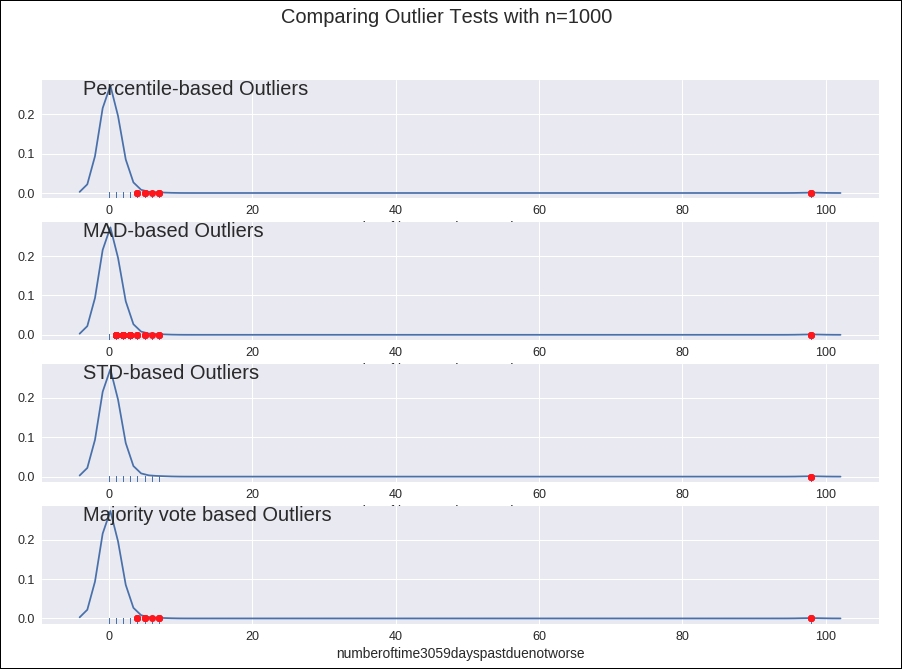Figure 1.28: Outlier detection graph

Refer to the frequency analysis of the data in the following figure: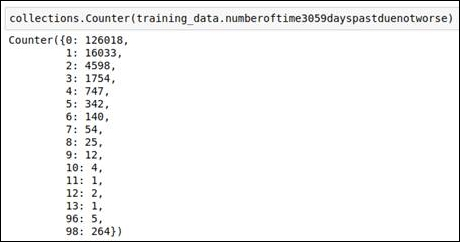Figure 1.29: Outlier values from the frequency calculation

The code snippet for replacing the outlier values with the median is given in the following figure: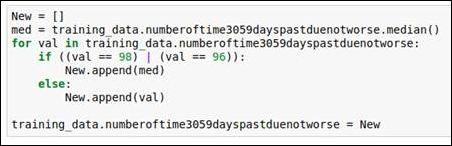Figure 1.30: Code snippet for replacing outliers

##### Debt ratio

If we look at the graph of the outlier detection of this attribute, then it's kind of confusing. Refer to the following figure: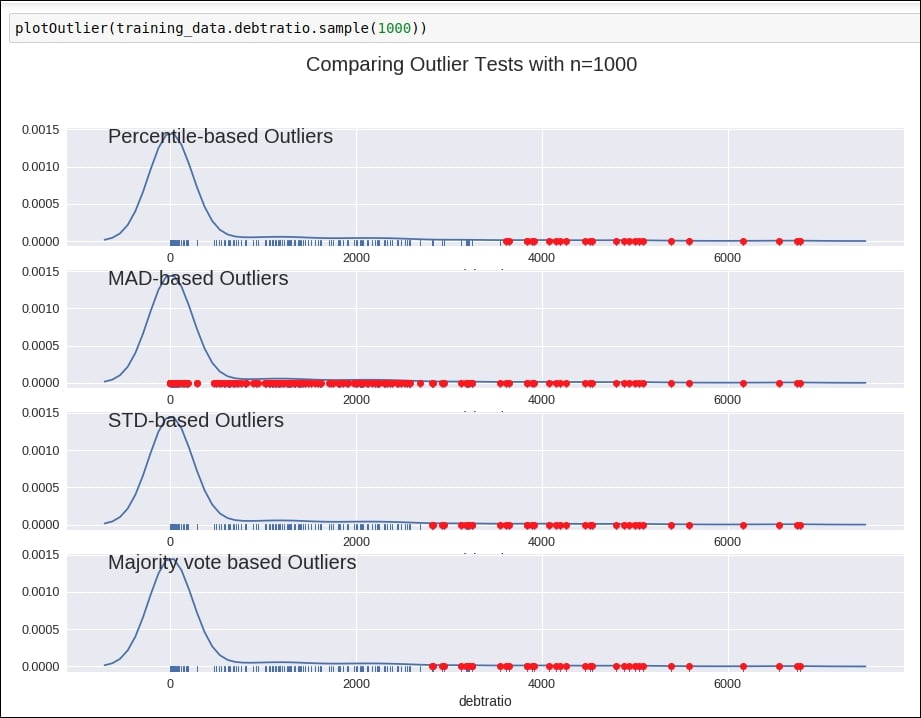Figure 1.31: Graph of outlier detection for the debt ratio column

Why? It's confusing because we are not sure which outlier detection method we should consider. So, here, we do some comparative analysis just by counting the number of outliers derived from each of the methods. Refer to the following figure: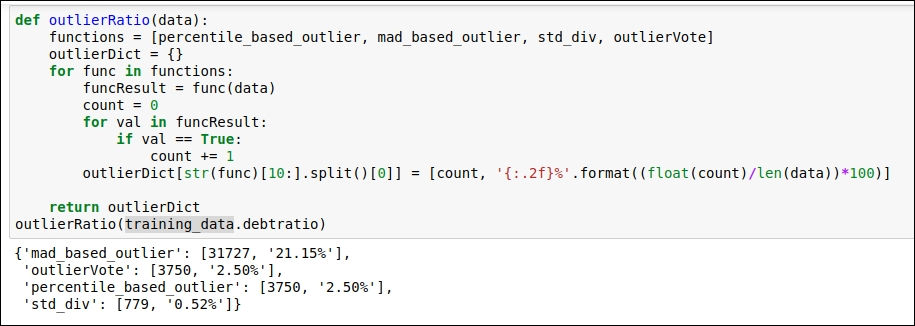Figure 1.32: Comparison of various outlier detection techniques

The maximum number of outliers was detected by the MAD-based method, so we will consider that method. Here, we will find the minimum upper bound value in order to replace the outlier values. The minimum upper bound is the minimum value derived from the outlier value. Refer to the following code snippet: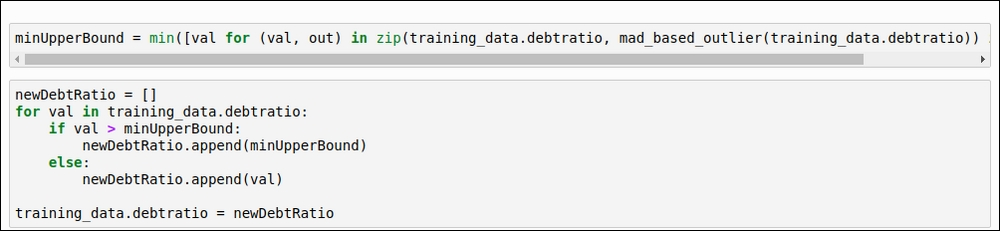Figure 1.33: The code for the minimum upper bound

##### Monthly income

For this data attribute, we will select the voting-based outlier detection method, as shown in the following figure: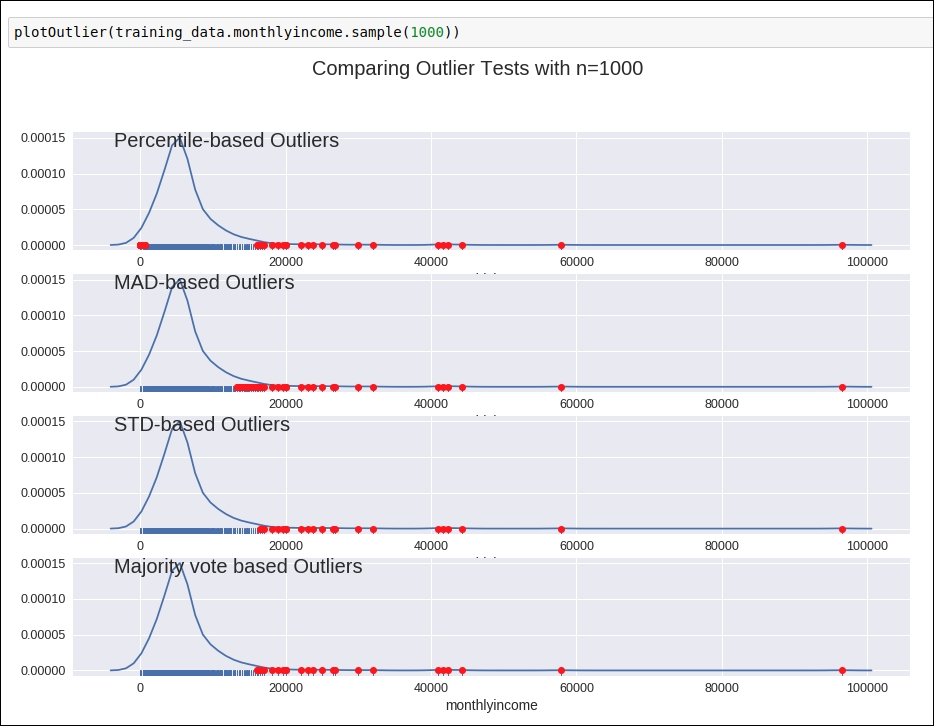Figure 1.34: Outlier detection graph

In order to replace the outlier, we will use the same logic that we have for the `debt ratio` data attribute. We replace the outliers by generating a minimum upper bound value. You can refer to the code given in the following figure: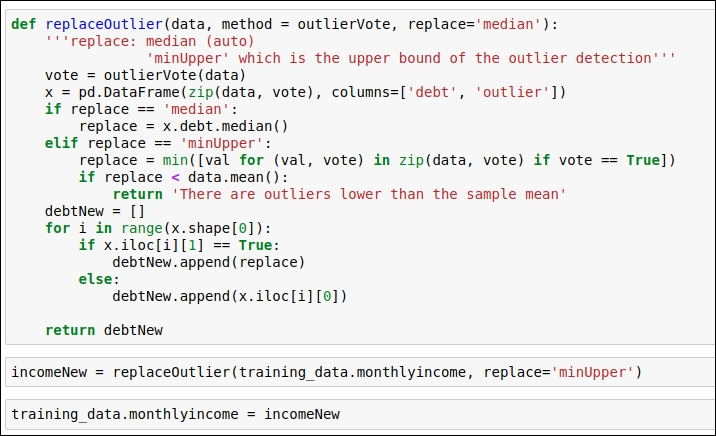Figure 1.35: Replace the outlier value with the minimum upper bound value

##### Number of open credit lines and loans

If you refer to the graph given in the following figure, you will see that there are no highly deviated outlier values present in this column: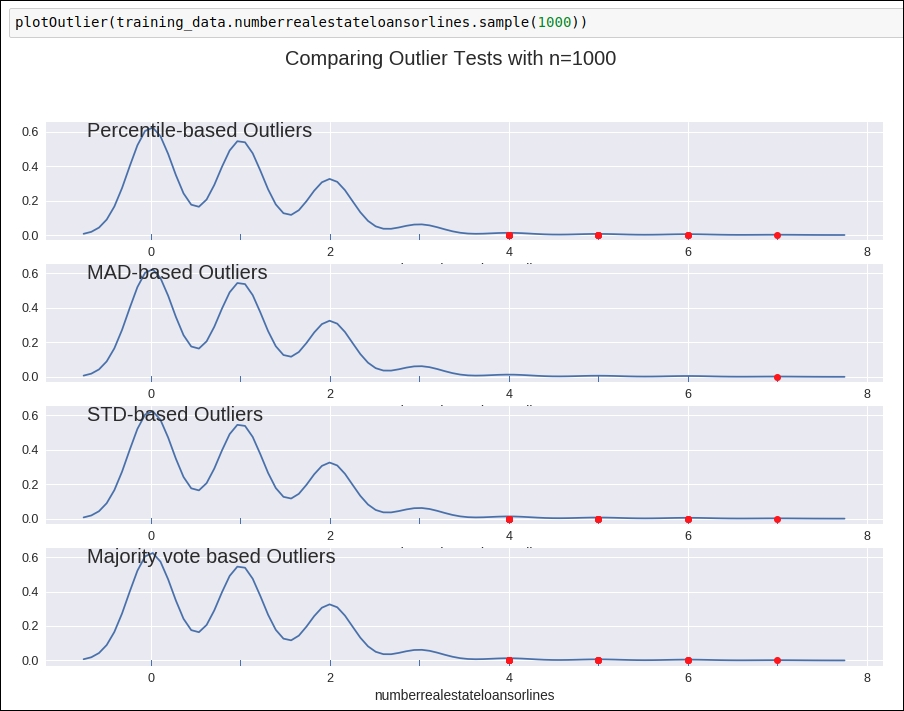Figure 1.36: Outlier detection graph

So, we will not perform any kind of replacement operation for this data attribute.

##### Number of times 90 days late

For this attribute, when you analyze the data value frequency, you will immediately see that the values 96 and 98 are outliers. We will replace these values with the median value of the data attribute.

Refer to the frequency analysis code snippet in the following figure: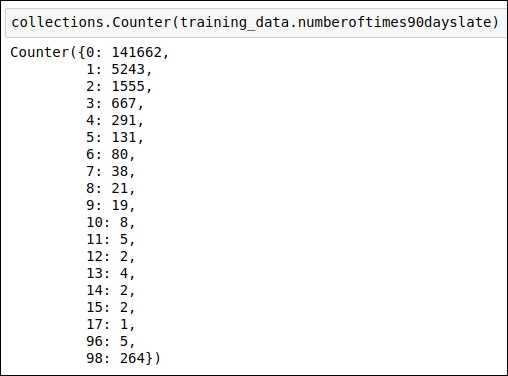Figure 1.37: Frequency analysis of the data points

The outlier replacement code snippet is shown in the following figure: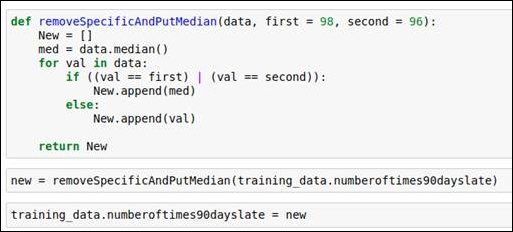Figure 1.38: Outlier replacement using the median value

##### Number of real estate loans or lines

When we see the frequency of value present in the data attribute, we will come to know that a frequency value beyond 17 is too less. So, here we replace every value less than 17 with 17.

You can refer to the code snippet in the following figure: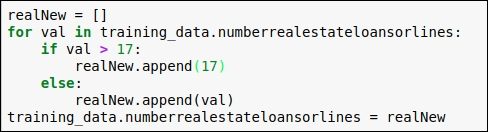Figure 1.39: Code snippet for replacing outliers

##### Number of times 60-89 days past due not worse

For this attribute, when you analyze the data value frequency, you will immediately see that the values 96 and 98 are outliers. We will replace these values with the median value of the data attribute.

Refer to the frequency analysis code snippet in the following figure: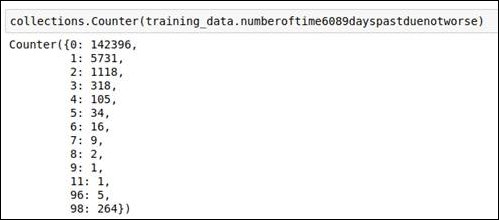Figure 1.40: Frequency analysis of the data

The outlier replacement code snippet is shown in the following figure: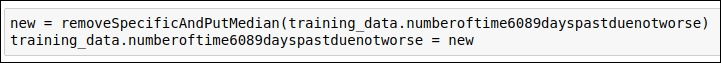Figure 1.41: Code snippet for replacing outliers using the median value

You can refer to the `removeSpecificAndPutMedian` method code from Figure 1.38.

#### Number of dependents

For this attribute, when you see the frequency value of the data points, you will immediately see that data values greater than 10 are outliers. We replace values greater than 10 with 10.

Refer to the code snippet in the following figure: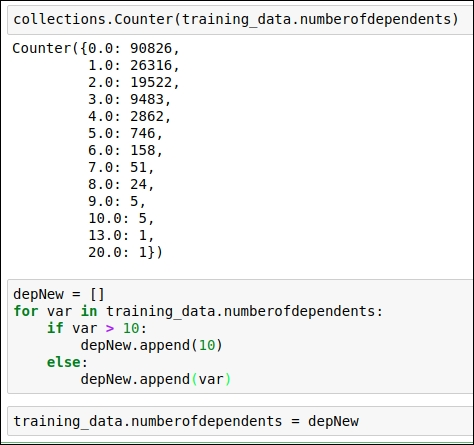Figure 1.42: Code snippet for replacing outlier values

This is the end of the outlier section. In this section, we've replaced the value of the data points in a more meaningful way. We have also reached the end of our basic data analysis section. This analysis has given us a good understanding of the dataset and its values. The next section is all about feature engineering. So, we will start with the basics first, and later on in this chapter, you will learn how feature engineering will impact the accuracy of the algorithm in a positive manner.

## Feature engineering for the baseline model

In this section, you will learn how to select features that are important in order to develop the predictive model. So right now, just to begin with, we won't focus much on deriving new features at this stage because first, we need to know which input variables / columns / data attributes / features give us at least baseline accuracy. So, in this first iteration, our focus is on the selection of features from the available training dataset.

### Finding out Feature importance

We need to know which the important features are. In order to find that out, we are going to train the model using the Random Forest classifier. After that, we will have a rough idea about the important features for us. So let's get straight into the code. You can refer to the code snippet in the following figure: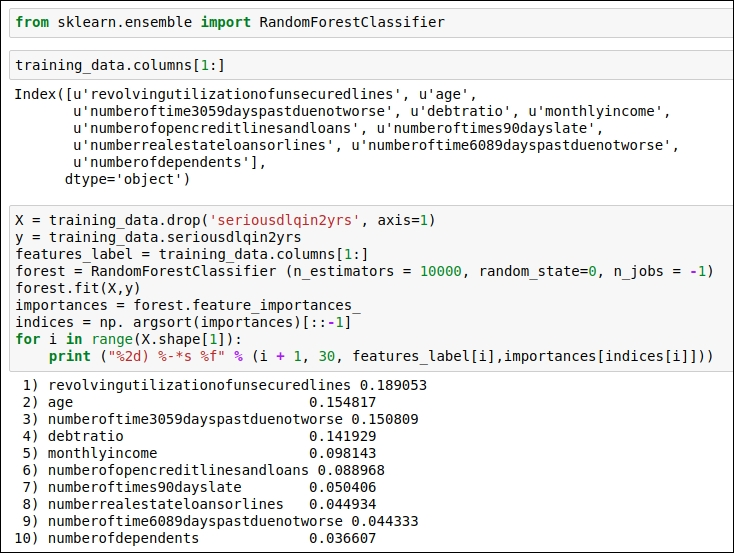Figure 1.43: Derive the importance of features

In this code, we are using Random Forest Classifier from scikit-learn. We use the `fit()` function to perform training, and then, in order to generate the importance of the features, we will use the `feature_importances`_ function, which is available in the scikit-learn library. Then, we will print the features with the highest importance value to the lowest importance value.

Let's draw a graph of this to get a better understanding of the most important features. You can find the code snippet in the following figure: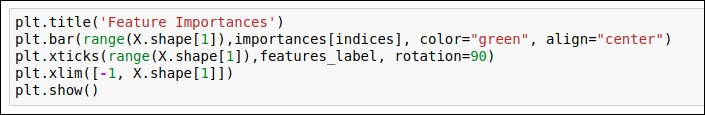Figure 1.44: Code snippet for generating a graph for feature importance

In this code snippet, we are using the matplotlib library to draw the graph. Here, we use a bar graph and feed in the values of all the data attributes and their importance values, which we previously derived. You can refer to the graph in the following figure: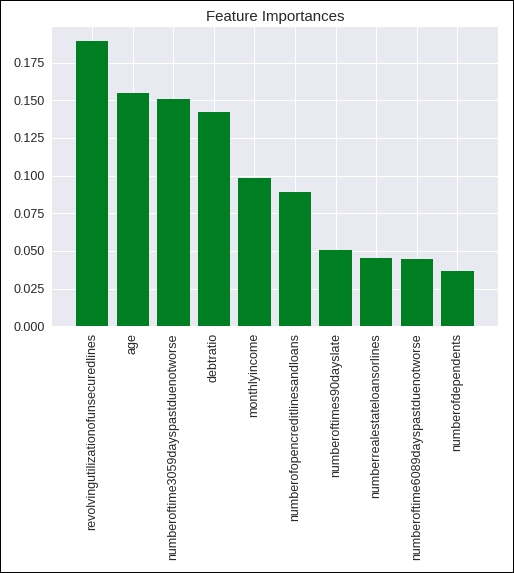Figure 1.45: Graph of feature importance

For the first iteration, we did this quite some work on the feature engineering front. We will surely revisit feature engineering in the upcoming sections. Now it's time to implement machine learning algorithms to generate the baseline predictive model, which will give us an idea of whether a person will default on a loan in the next 2 years or not. So let's jump to the next section.

## Selecting machine learning algorithms

This section is the most important one. Here, we will try a couple of different ML algorithms in order to get an idea about which ML algorithm performs better. Also, we will perform a training accuracy comparison.

By this time, you will definitely know that this particular problem is considered a classification problem. The algorithms that we are going to choose are as follows (this selection is based on intuition):

• K-Nearest Neighbor (KNN)

• Logistic Regression

• RandomForest

Our first step is to generate the training data in a certain format. We are going to split the training dataset into a training and testing dataset. So, basically, we are preparing the input for our training. This is common for all the ML algorithms. Refer to the code snippet in the following figure: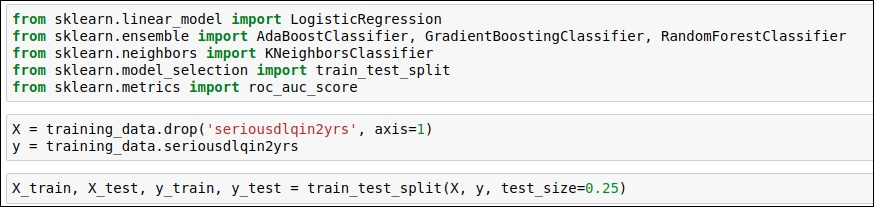Figure 1.46: Code snippet for generating a training dataset in the key-value format for training

As you can see in the code, variable x contains all the columns except the target column entitled `seriousdlqin2yrs`, so we have dropped this column. The reason behind dropping this attribute is that this attribute contains the answer/target/label for each row. ML algorithms need input in terms of a key-value pair, so a target column is key and all other columns are values. We can say that a certain pattern of values will lead to a particular target value, which we need to predict using an ML algorithm.

Here, we also split the training data. We will use 75% of the training data for actual training purposes, and once training is completed, we will use the remaining 25% of the training data to check the training accuracy of our trained ML model. So, without wasting any time, we will jump to the coding of the ML algorithms, and I will explain the code to you as and when we move forward. Note that here, I'm not get into the mathematical explanation of the each ML algorithm but I am going to explain the code.

### K-Nearest Neighbor (KNN)

In this algorithm, generally, our output prediction follows the same tendency as that of its neighbor. K is the number of neighbors that we are going to consider. If K=3, then during the prediction output, check the three nearest neighbor points, and if one neighbor belongs to X category and two neighbors belongs to Y category, then the predicted label will be Y, as the majority of the nearest points belongs to the Y category.

Let's see what we have coded. Refer to the following figure: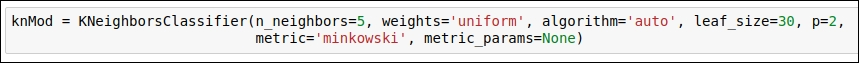Figure 1.47: Code snippet for defining the KNN classifier

Let's understand the parameters one by one:

• As per the code, K=5 means our prediction is based on the five nearest neighbors. Here, `n_neighbors=5`.

• Weights are selected uniformly, which means all the points in each neighborhood are weighted equally. Here, weights='uniform'.

• algorithm='auto': This parameter will try to decide the most appropriate algorithm based on the values we passed.

• leaf_size = 30: This parameter affects the speed of the construction of the model and query. Here, we have used the default value, which is 30.

• p=2: This indicates the power parameter for the Minkowski metric. Here, p=2 uses `euclidean_distance`.

• metric='minkowski': This is the default distance metric, which helps us build the tree.

• metric_params=None: This is the default value that we are using.

### Logistic regression

Logistic regression is one of most widely used ML algorithms and is also one of the oldest. This algorithm generates probability for the target variable using sigmod and other nonlinear functions in order to predict the target labels.

Let's refer to the code and the parameter that we have used for Logistic regression. You can refer to the code snippet given in the following figure: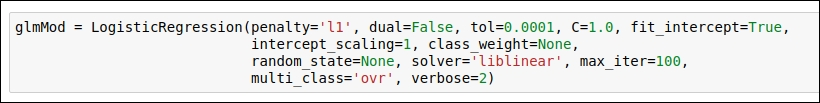Figure 1.48: Code snippet for the Logistic regression ML algorithm

Let's understand the parameters one by one:

• penalty='l1': This parameter indicates the choice of the gradient descent algorithm. Here, we have selected the Newton-Conjugate_Gradient method.

• dual=False: If we have number of sample > number of features, then we should set this parameter as false.

• tol=0.0001: This is one of the stopping criteria for the algorithm.

• c=1.0: This value indicates the inverse of the regularization strength. This parameter must be a positive float value.

• fit_intercept = True: This is a default value for this algorithm. This parameter is used to indicate the bias for the algorithm.

• solver='liblinear': This algorithm performs well for small datasets, so we chose that.

• intercept_scaling=1: If we select the liblinear algorithm and fit_intercept = True, then this parameter helps us generate the feature weight.

• class_weight=None: There is no weight associated with the class labels.

• random_state=None: Here, we use the default value of this parameter.

• max_iter=100: Here, we iterate 100 times in order to converge our ML algorithm on the given dataset.

• multi_class='ovr': This parameter indicates that the given problem is the binary classification problem.

• verbose=2: If we use the liblinear in the solver parameter, then we need to put in a positive number for verbosity.

This particular algorithm has N number of iterations. In the first iteration, we start by taking random data points from the training dataset and building the model. After each iteration, the algorithm checks for data points in which the classifier doesn't perform well. Once those data points are identified by the algorithm based on the error rate, the weight distribution is updated. So, in the next iteration, there are more chances that the algorithm will select the previously poorly classified data points and learn how to classify them. This process keeps running for the given number of iterations you provide.

Let's refer to the code snippet given in the following figure: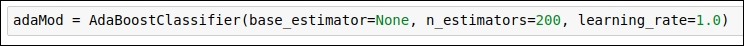Figure 1.49: Code snippet for the AdaBosst classifier

The parameter-related description is given as follows:

• base_estimator = None: The base estimator from which the boosted ensemble is built.

• n_estimators=200: The maximum number of estimators at which boosting is terminated. After 200 iterations, the algorithm will be terminated.

• learning_rate=1.0: This rate decides how fast our model will converge.

This algorithm is also a part of the ensemble of ML algorithms. In this algorithm, we use basic regression algorithm to train the model. After training, we will calculate the error rate as well as find the data points for which the algorithm does not perform well, and in the next iteration, we will take the data points that introduced the error and retrain the model for better prediction. The algorithm uses the already generated model as well as a newly generated model to predict the values for the data points.

You can see the code snippet in the following figure: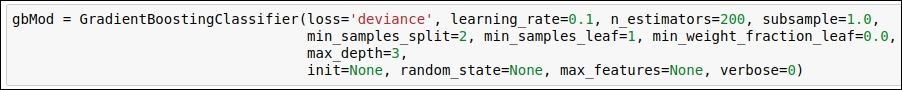Figure 1.50: Code snippet for the Gradient Boosting classifier

Let's go through the parameters of the classifier:

• loss='deviance': This means that we are using logistic regression for classification with probabilistic output.

• learning_rate = 0.1: This parameter tells us how fast the model needs to converge.

• n_estimators = 200: This parameter indicates the number of boosting stages that are needed to be performed.

• subsample = 1.0: This parameter helps tune the value for bias and variance. Choosing subsample < 1.0 leads to a reduction in variance and an increase in bias.

• min_sample_split=2: The minimum number of samples required to split an internal node.

• min_weight_fraction_leaf=0.0: Samples have equal weight, so we have provided the value 0.

• max_depth=3: This indicates the maximum depth of the individual regression estimators. The maximum depth limits the number of nodes in the tree.

• init=None: For this parameter, loss.init_estimator is used for the initial prediction.

• random_state=None: This parameter indicates that the random state is generated using the `numpy.random` function.

• max_features=None: This parameter indicates that we have N number of features. So, `max_features=n_features`.

• verbose=0: No progress has been printed.

### RandomForest

This particular ML algorithm generates the number of decision trees and uses the voting mechanism to predict the target label. In this algorithm, there are a number of decision trees generated, creating a forest of trees, so it's called RandomForest.

In the following code snippet, note how we have declared the RandomForest classifier: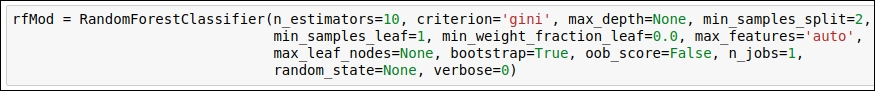Figure 1.51: Code snippet for Random Forest Classifier

Let's understand the parameters here:

• `n_estimators=10`: This indicates the number of trees in the forest.

• `criterion='gini'`: Information gained will be calculated by gini.

• `max_depth=None`: This parameter indicates that nodes are expanded until all leaves are pure or until all leaves contain less than min_samples_split samples.

• `min_samples_split=2`: This parameter indicates that there is a minimum of two samples required to perform splitting in order to generate the tree.

• `min_samples_leaf=1`: This indicates the sample size of the leaf node.

• `min_weight_fraction_leaf=0.0`: This parameter indicates the minimum weighted fraction of the sum total of weights (of all the input samples) required to be at a leaf node. Here, weight is equally distributed, so a sample weight is zero.

• `max_features='auto'`: This parameter is considered using the auto strategy. We select the auto value, and then we select max_features=sqrt(n_features).

• `max_leaf_nodes=None`: This parameter indicates that there can be an unlimited number of leaf nodes.

• `bootstrap=True`: This parameter indicates that the bootstrap samples are used when trees are being built.

• `oob_score=False`: This parameter indicates whether to use out-of-the-bag samples to estimate the generalization accuracy. We are not considering out-of-the-bag samples here.

• `n_jobs=1`: Both fit and predict job can be run in parallel if `n_job = 1`.

• `random_state=None`: This parameter indicates that random state is generated using the `numpy.random` function.

• `verbose=0`: This controls the verbosity of the tree building process. 0 means we are not printing the progress.

Up until now, we have seen how we declare our ML algorithm. We have also defined some parameter values. Now, it's time to train this ML algorithm on the training dataset. So let's discuss that.

## Training the baseline model

In this section, we will perform actual training using the following ML algorithms. This step is time-consuming as it needs more computation power. We use 75% of the training dataset for actual training and 25% of the dataset for testing in order to measure the training accuracy.

You can find the code snippet in the following figure: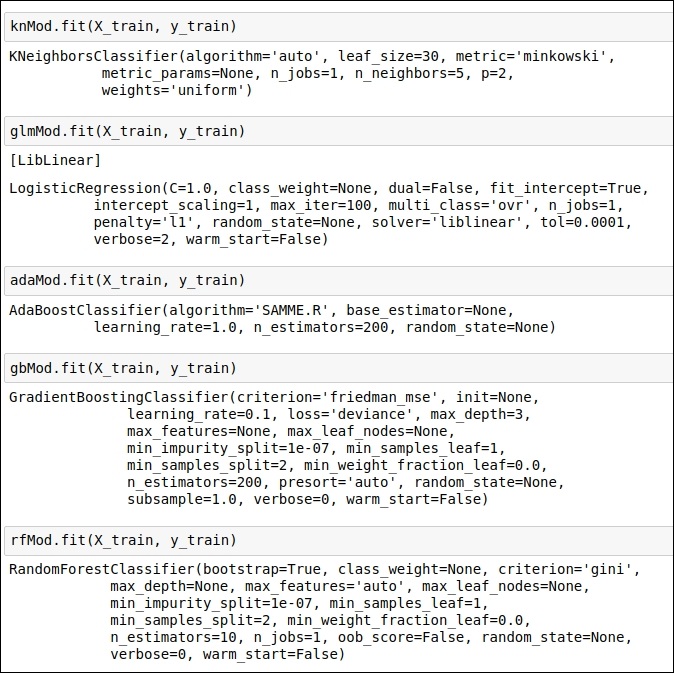Figure 1.52: Code snippet for performing training

In the preceding code snippet, you can see that we performed the actual training operation using the `fit()` function from the scikit-learn library. This function uses the given parameter and trains the model by taking the input of the target data attribute and other feature columns.

Once you are done with this step, you'll see that our different ML algorithms generate different trained models. Now it's time to check how good our trained model is when it comes to prediction. There are certain techniques that we can use on 25% of the dataset. In the next section, we will understand these techniques.

## Understanding the testing matrix

In this section, we will look at some of the widely used testing matrices that we can use in order to get an idea about how good or bad our trained model is. This testing score gives us a fair idea about which model achieves the highest accuracy when it comes to the prediction of the 25% of the data.

Here, we are using two basic levels of the testing matrix:

• The mean accuracy of the trained models

• The ROC-AUC score

### The Mean accuracy of the trained models

In this section, we will understand how scikit-learn calculates the accuracy score when we use the scikit-learn function `score()` to generate the training accuracy. The function score() returns the mean accuracy. More precisely, it uses residual standard error. Residual standard error is nothing but the positive square root of the mean square error. Here, the equation for calculating accuracy is as follows: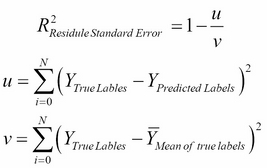The best possible score is 1.0 and the model can have a negative score as well (because the model can be arbitrarily worse). If a constant model always predicts the expected value of y, disregarding the input features, it will get a residual standard error score of 0.0.

### The ROC-AUC score

The ROC-AUC score is used to find out the accuracy of the classifier. ROC and AUC are two different terms. Let's understand each of the terms one by one.

#### ROC

ROC stands for Receiver Operating Characteristic. It's is a type of curve. We draw the ROC curve to visualize the performance of the binary classifier. Now that I have mentioned that ROC is a curve, you may want to know which type of curve it is, right? The ROC curve is a 2-D curve. It's x axis represents the False Positive Rate (FPR) and its y axis represents the True Positive Rate (TPR). TPR is also known as sensitivity, and FPR is also known as specificity (SPC). You can refer to the following equations for FPR and TPR.

TPR = True Positive / Number of positive samples = TP / P

FPR = False Positive / Number of negative samples = FP / N = 1 - SPC

For any binary classifier, if the predicted probability is ≥ 0.5, then it will get the class label X, and if the predicted probability is < 0.5, then it will get the class label Y. This happens by default in most binary classifiers. This cut-off value of the predicted probability is called the threshold value for predictions. For all possible threshold values, FPR and TPR have been calculated. This FPR and TPR is an x,y value pair for us. So, for all possible threshold values, we get the x,y value pairs, and when we put the points on an ROC graph, it will generate the ROC curve. If your classifier perfectly separates the two classes, then the ROC curve will hug the upper-right corner of the graph. If the classifier performance is based on some randomness, then the ROC curve will align more to the diagonal of the ROC curve. Refer to the following figure: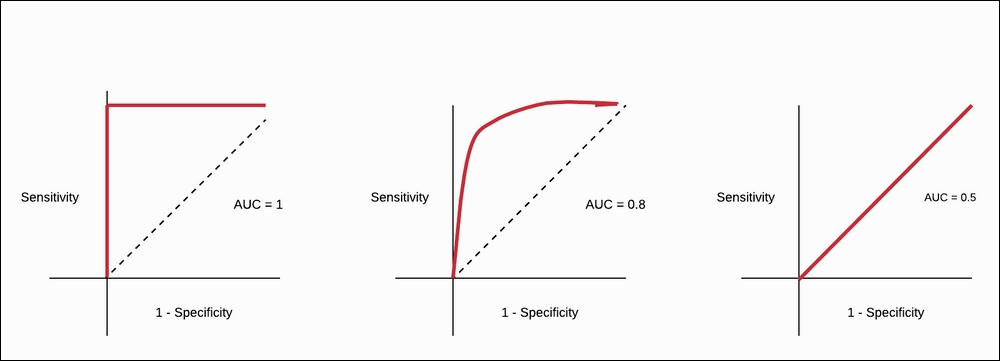Figure 1.53: ROC curve for different classification scores

In the preceding figure, the leftmost ROC curve is for the perfect classifier. The graph in the center shows the classifier with better accuracy in real-world problems. The classifier that is very random in its guess is shown in the rightmost graph. When we draw an ROC curve, how can we quantify it? In order to answer that question, we will introduce AUC.

#### AUC

AUC stands for Area Under the Curve. In order to quantify the ROC curve, we use the AUC. Here, we will see how much area has been covered by the ROC curve. If we obtain a perfect classifier, then the AUC score is 1.0, and if we have a classifier that is random in its guesses, then the AUC score is 0.5. In the real world, we don't expect an AUC score of 1.0, but if the AUC score for the classifier is in the range of 0.6 to 0.9, then it will be considered a good classifier. You can refer to the following figure: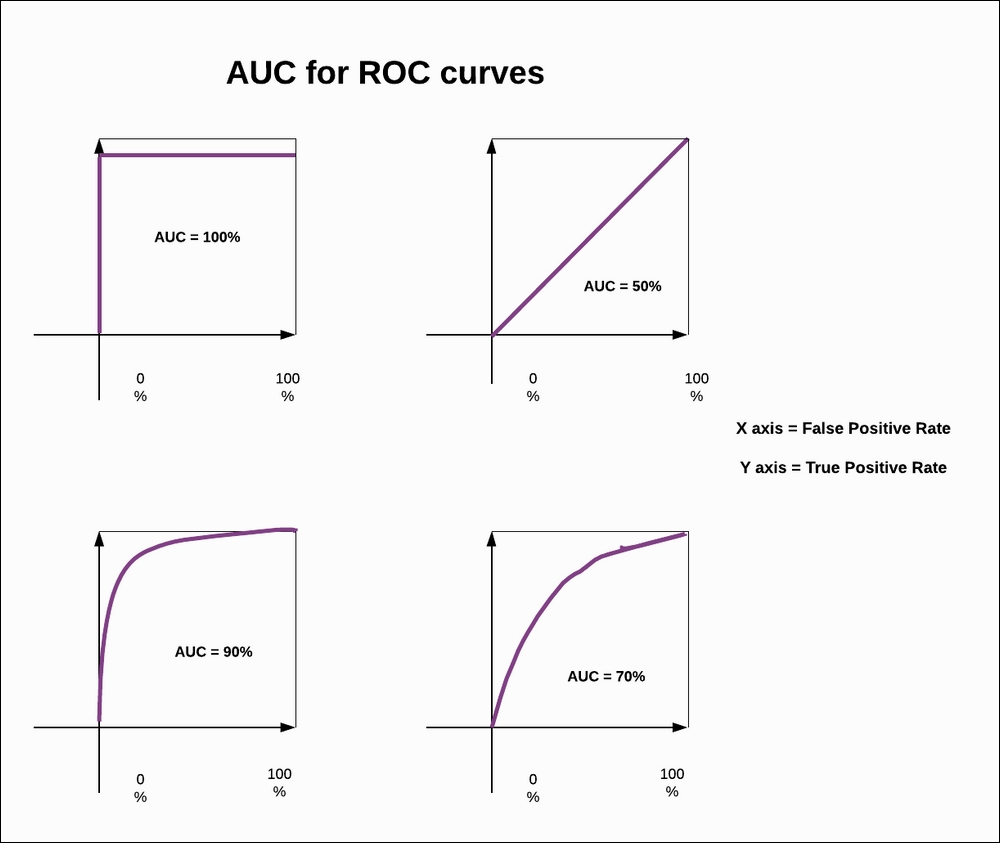Figure 1.54: AUC for the ROC curve

In the preceding figure, you can see how much area under the curve has been covered, and that becomes our AUC score. This gives us an indication of how good or bad our classifier is performing.

These are the two matrices that we are going to use. In the next section, we will implement actual testing of the code and see the testing matrix for our trained ML models.

## Testing the baseline model

In this section, we will implement the code, which will give us an idea about how good or how bad our trained ML models perform in a validation set. We are using the mean accuracy score and the AUC-ROC score.

Here, we have generated five different classifiers and, after performing testing for each of them on the validation dataset, which is 25% of held-out dataset from the training dataset, we will find out which ML model works well and gives us a reasonable baseline score. So let's look at the code:.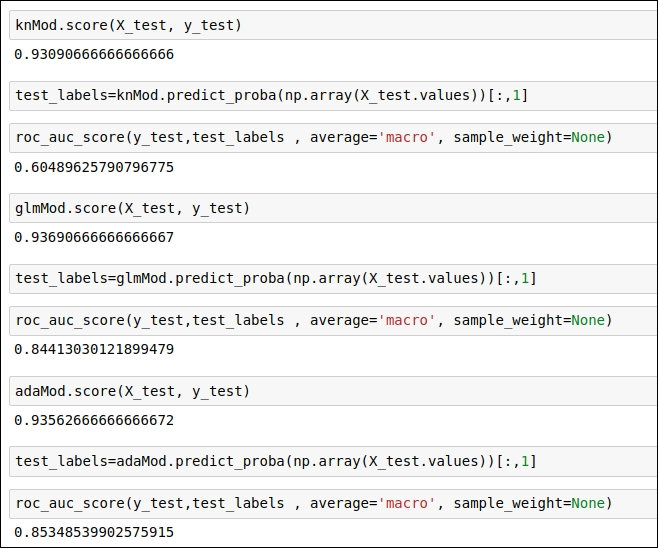Figure 1.55: Code snippet to obtain a test score for the trained ML model

In the preceding code snippet, you can see the scores for three classifiers.

Refer to the code snippet in the following figure: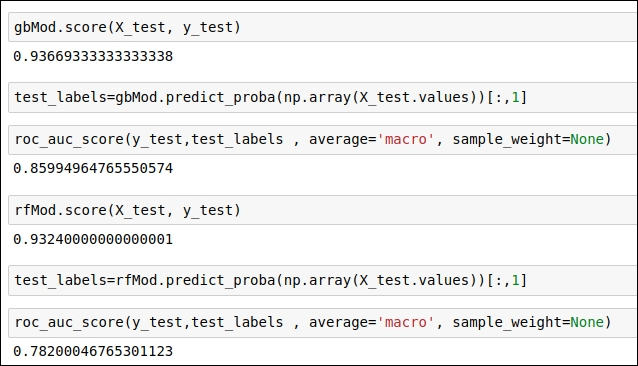Figure 1.56: Code snippet to obtain the test score for the trained ML model

In the code snippet, you can see the score of the two classifiers.

Using the `score()` function of scikit-learn, you will get the mean accuracy score, whereas, the `roc_auc_score()` function will provide you with the ROC-AUC score, which is more significant for us because the mean accuracy score considers only one threshold value, whereas the ROC-AUC score takes into consideration all possible threshold values and gives us the score.

As you can see in the code snippets given above, the AdaBoost and GradientBoosting classifiers get a good ROC-AUC score on the validation dataset. Other classifiers, such as logistic regression, KNN, and RandomForest do not perform well on the validation set. From this stage onward, we will work with AdaBoost and GradientBoosting classifiers in order to improve their accuracy score.

In the next section, we will see what we need to do in order to increase classification accuracy. We need to list what can be done to get good accuracy and what are the current problems with the classifiers. So let's analyze the problem with the existing classifiers and look at their solutions.

## Problems with the existing approach

We got the baseline score using the AdaBoost and GradientBoosting classifiers. Now, we need to increase the accuracy of these classifiers. In order to do that, we first list all the areas that can be improvised but that we haven't worked upon extensively. We also need to list possible problems with the baseline approach. Once we have the list of the problems or the areas on which we need to work, it will be easy for us to implement the revised approach.

Here, I'm listing some of the areas, or problems, that we haven't worked on in our baseline iteration:

• Problem: We haven't used cross-validation techniques extensively in order to check the overfitting issue.

• Solution: If we use cross-validation techniques properly, then we will know whether our trained ML model suffers from overfitting or not. This will help us because we don't want to build a model that can't even be generalized properly.

• Problem: We also haven't focused on hyperparameter tuning. In our baseline approach, we mostly use the default parameters. We define these parameters during the declaration of the classifier. You can refer to the code snippet given in Figure 1.52, where you can see the classifier taking some parameters that are used when it trains the model. We haven't changed these parameters.

• Solution: We need to tune these hyperparameters in such a way that we can increase the accuracy of the classifier. There are various hyperparameter-tuning techniques that we need to use.

In the next section, we will look at how these optimization techniques actually work as well as discuss the approach that we are going to take. So let's begin!

## Optimizing the existing approach

In this section, we will gain an understanding of the basic technicality regarding cross-validation and hyperparameter tuning. Once we understand the basics, it will be quite easy for us to implement them. Let's start with a basic understanding of cross-validation and hyperparameter tuning.

### Understanding key concepts to optimize the approach

In this revised iteration, we need to improve the accuracy of the classifier. Here, we will cover the basic concepts first and then move on to the implementation part. So, we will understand two useful concepts:

• Cross-validation

• Hyperparameter tuning

#### Cross-validation

Cross-validation is also referred to as rotation estimation. It is basically used to track a problem called overfitting. Let me start with the overfitting problem first because the main purpose of using cross-validation is to avoid the overfitting situation.

Basically, when you train the model using the training dataset and check its accuracy, you find out that your training accuracy is quite good, but when you apply this trained model on an as-yet-unseen dataset, you realize that the trained model does not perform well on the unseen dataset and just mimics the output of the training dataset in terms of its target labels. So, we can say that our trained model is not able to generalize properly. This problem is called overfitting, and in order to solve this problem, we need to use cross-validation.

In our baseline approach, we didn't use cross-validation techniques extensively. The good part is that, so far, we generated our validation set of 25% of the training dataset and measured the classifier accuracy on that. This is a basic technique used to get an idea of whether the classifier suffers from overfitting or not.

There are many other cross validation techniques that will help us with two things:

• Tracking the overfitting situation using CV: This will give us a perfect idea about the overfitting problem. We will use K-fold CV.

• Model selection using CV: Cross-validation will help us select the classification models. This will also use K-fold CV.

Now let's look at the single approach that will be used for both of these tasks. You will find the implementation easy to understand.

##### The approach of using CV

The scikit-learn library provides great implementation of cross-validation. If we want to implement cross-validation, we just need to import the cross-validation module. In order to improvise on accuracy, we will use K-fold cross-validation. What this K-fold cross-validation basically does is explained here.

When we use the train-test split, we will train the model by using 75% of the data and validate the model by using 25% of the data. The main problem with this approach is that, actually, we are not using the whole training dataset for training. So, our model may not be able to come across all of the situations that are present in the training dataset. This problem has been solved by K-fold CV.

In K-fold CV, we need to provide the positive integer number for K. Here, you divide the training dataset into the K sub-dataset. Let me give you an example. If you have 125 data records in your training dataset and you set the value as k = 5, then each subset of the data gets 25 data records. So now, we have five subsets of the training dataset with 25 records each.

Let's understand how these five subsets of the dataset will be used. Based on the provided value of K, it will be decided how many times we need to iterate over these subsets of the data. Here we have taken K=5. So, we iterate over the dataset K-1 = 5-1 =4 times. Note that the number of iterations in K-fold CV is calculated by the equation K-1. Now let's see what happens to each of the iterations:

• First iteration: We take one subset for testing and the remaining four subsets for training.

• Second iteration: We take two subsets for testing and the remaining three subsets for training.

• Third iteration: We take three subsets for testing and the remaining two subsets for training.

• Fourth iteration: We take four subsets for testing and the remaining subset for training. After this fourth iteration, we don't have any subsets left for training or testing, so we stop after iteration K-1.

This approach has the following advantages:

K-fold CV uses all the data points for training, so our model takes advantage of getting trained using all of the data points.

• After every iteration, we get the accuracy score. This will help us decide how models perform.

• We generally consider the mean value and standard deviation value of the cross-validation after all the iterations have been completed. For each iteration, we track the accuracy score, and once all iterations have been done, we take the mean value of the accuracy score as well as derive the standard deviation (std) value from the accuracy scores. This CV mean and standard deviation score will help us identify whether the model suffers from overfitting or not.

• If you perform this process for multiple algorithms then based on this mean score and the standard score, you can also decide which algorithm works best for the given dataset.

The disadvantage of this approach is as follows:

• This k-fold CV is a time-consuming and computationally expensive method.

So after reading this, you hopefully understand the approach and, by using this implementation, we can ascertain whether our model suffers from overfitting or not. This technique will also help us select the ML algorithm. We will check out the implementation of this in the Implementing the Revised Approach section.

Now let's check out the next optimization technique, which is hyperparameter tuning.

#### Hyperparameter tuning

In this section, we will look at how we can use a hyperparameter-tuning technique to optimize the accuracy of our model. There are some kind of parameters whose value cannot be learnt during training process. These parameters are expressing higher-level properties of the ML model. These higher-level parameters are called hyperparameters. These are tuning nobs for ML model. We can obtain the best value for hyperparameter by trial and error. You can refer more on this by using this link: https://machinelearningmastery.com/difference-between-a-parameter-and-a-hyperparameter/, If we come up with the optimal value of the hyperparameters, then we will able to achieve the best accuracy for our model, but the challenging part is that we don't know the exact values of these parameters over our head. These parameters are the tuning knobs for our algorithm. So, we need to apply some techniques that will give us the best possible value for our hyperparameter, which we can use when we perform training.

In scikit-learn, there are two functions that we can use in order to find these hyperparameter values, which are as follows:

• Grid search parameter tuning

• Random search parameter tuning

##### Grid search parameter tuning

In this section, we will look at how grid search parameter tuning works. We specify the parameter values in a list called grid. Each value specified in grid has been taken in to consideration during the parameter tuning. . The model has been built and evaluated based on the specified grid value. This technique exhaustively considers all parameter combinations and generates the final optimal parameters.

Suppose we have five parameters that we want to optimize. Using this technique, if we want to try 10 different values for each of the parameters, then it will take 105 evaluations. Assume that, on average, for each parameter combination, 10 minutes are required for training; then, for the evaluation of 105, it will take years. Sounds crazy, right? This is the main disadvantage of this technique. This technique is very time consuming. So, a better solution is random search. '

##### Random search parameter tuning

The intuitive idea is the same as grid search, but the main difference is that instead of trying out all possible combinations, we will just randomly pick up the parameter from the selected subset of the grid. If I want to add on to my previous example, then in random search, we will take a random subset value of the parameter from 105 values. Suppose that we take only 1,000 values from 105 values and try to generate the optimal value for our hyperparameters. This way, we will save time.

In the revised approach, we will use this particular technique to optimize the hyperparameters.

From the next section, we will see the actual implementation of K-fold cross-validation and hyperparameter tuning. So let's start implementing our approach.

## Implementing the revised approach

In this section, we will see the actual implementation of our revised approach, and this revised approach will use K-fold cross-validation and hyperparameter optimization. I have divided the implementation part into two sections so you can connect the dots when you see the code. The two implementation parts are as follows:

• Implementing a cross-validation based approach

• Implementing hyperparameter tuning

### Implementing a cross-validation based approach

In this section, we will see the actual implementation of K-fold CV. Here, we are using the scikit-learn cross-validation score module. So, we need to choose the value of K-fold. By default, the value is 3. I'm using the value of K = 5. You can refer to the code snippet given in the following figure: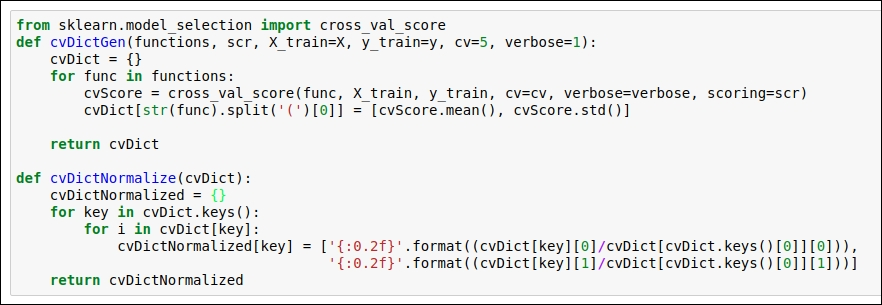Figure 1.57: Code snippet for the implementation of K-fold cross validation

As you can see in the preceding figure, we obtain `cvScore.mean()` and `cvScore.std()` scores to evaluate our model performance. Note that we have taken the whole training dataset into consideration. So, the values for these parameters are `X_train = X` and `y_train = y`. Here, we define the `cvDictGen` function , which will track the mean value and the standard deviation of the accuracy. We have also implemented the `cvDictNormalize` function, which we can use if we want to obtain a normalized mean and a standard deviation (std) score. For the time being, we are not going to use the `cvDictNormalize` function.

Now it's time to run the `cvDictGen` method. You can see the output in the following figure: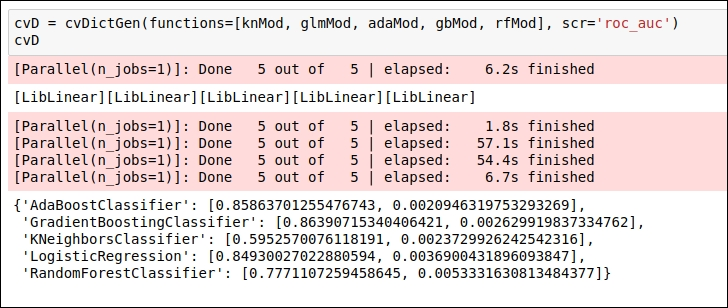Figure 1.58: Code snippet for the output of K-fold cross validation

We have performed cross-validation for five different ML algorithms to check which ML algorithm works well. As we can see, in our output given in the preceding figure, GradietBoosting and Adaboot classifier work well. We have used the cross-validation score in order to decide which ML algorithm we should select and which ones we should not go with. Apart from that, based on the mean value and the std value, we can conclude that our ROC-AUC score does not deviate much, so we are not suffering from the overfitting issue.

Now it's time to see the implementation of hyperparameter tuning.

### Implementing hyperparameter tuning

In this section, we will look at how we can obtain optimal values for hyperparameters. Here, we are using the `RandomizedSearchCV` hyperparameter tuning method. We have implemented this method for the AdaBoost and GradientBossting algorithms. You can see the implementation of hyperparameter tuning for the Adaboost algorithm in the following figure: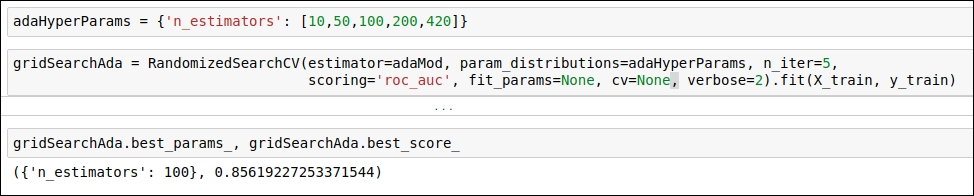Figure 1.59: Code snippet of hyperparameter tuning for the Adaboost algorithm

After running the `RandomizedSearchCV` method on the given values of parameters, it will generate the optimal parameter value. As you can see in the preceding figure, we want the optimal value for the parameter; `n_estimators`.`RandomizedSearchCV` obtains the optimal value for `n_estimators`, which is 100.

You can see the implementation of hyperparameter tuning for the GradientBoosting algorithm in the following figure: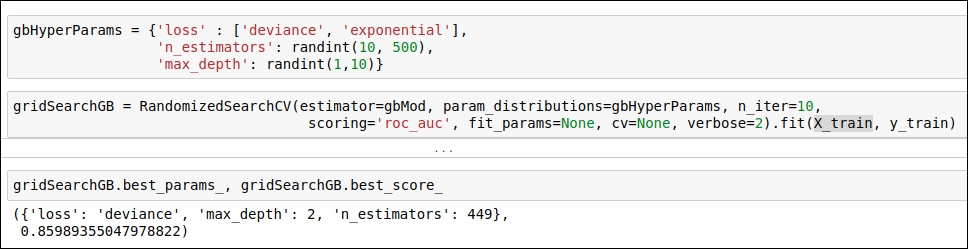Figure 1.60: Code snippet of hyperparameter tuning for the GradientBoosting algorithm

As you can see in the preceding figure, the `RandomizedSearchCV` method obtains the optimal value for the following hyperparameters:

• 'loss': 'deviance'

• 'max_depth': 2

• 'n_estimators': 449

Now it's time to test our revised approach. Let's see how we will test the model and what the outcome of the testing will be.

### Implementing and testing the revised approach

Here, we need to plug the optimal values of the hyperparameters, and then we will see the ROC-AUC score on the validation dataset so that we know whether there will be any improvement in the accuracy of the classifier or not.

You can see the implementation and how we have performed training using the best hyperparameters by referring to the following figure: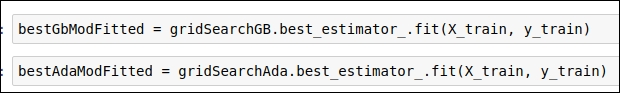Figure 1.61: Code snippet for performing training by using optimal hyperparameter values

Once we are done with the training, we can use the trained model to predict the target labels for the validation dataset. After that, we can obtain the ROC-AUC score, which gives us an idea of how much we are able to optimize the accuracy of our classifier. This score also helps validate our direction, so if we aren't able to improve our classifier accuracy, then we can identify the problem and improve accuracy in the next iteration. You can see the ROC-AUC score in the following figure: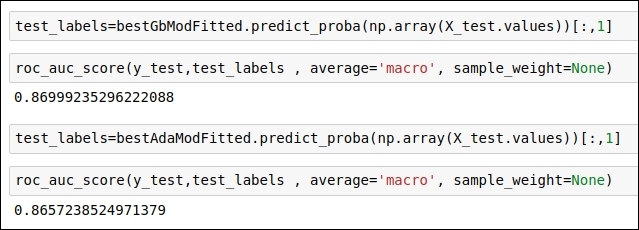Figure 1.62: Code snippet of the ROC-AUC score for the revised approach

As you can see in the output, after hyperparameter tuning, we have an improvement in the ROC-AUC score compared to our baseline approach. In our baseline approach, the ROC-AUC score for AdaBoost is 0.85348539, whereas after hyperparameter tuning, it is 0.86572352. In our baseline approach, the ROC-AUC score for GradientBoosting is 0.85994964, whereas after hyperparameter tuning, it is 0.86999235. These scores indicate that we are heading in the right direction.

The question remains: can we further improve the accuracy of the classifiers? Sure, there is always room for improvement, so we will follow the same approach. We list all the possible problems or areas we haven't touched upon yet. We try to explore them and generate the best possible approach that can give us good accuracy on the validation dataset as well as the testing dataset.

So let's see what our untouched areas in this revised approach will be.

### Understanding problems with the revised approach

Up until the revised approach, we did not spend a lot of time on feature engineering. So in our best possible approach, we spent time on the transformation of features engineering. We need to implement a voting mechanism in order to generate the final probability of the prediction on the actual test dataset so that we can get the best accuracy score.

These are the two techniques that we need to apply:

• Feature transformation

• An ensemble ML model with a voting mechanism

Once we implement these techniques, we will check our ROC-AUC score on the validation dataset. After that, we will generate a probability score for each of the records present in the real test dataset. Let's start with the implementation.

## Best approach

As mentioned in the previous section, in this iteration, we will focus on feature transformation as well as implementing a voting classifier that will use the AdaBoost and GradientBoosting classifiers. Hopefully, by using this approach, we will get the best ROC-AUC score on the validation dataset as well as the real testing dataset. This is the best possible approach in order to generate the best result. If you have any creative solutions, you can also try them as well. Now we will jump to the implementation part.

### Implementing the best approach

Here, we will implement the following techniques:

• Log transformation of features

• Voting-based ensemble model

Let's implement feature transformation first.

#### Log transformation of features

We will apply log transformation to our training dataset. The reason behind this is that we have some attributes that are very skewed and some data attributes that have values that are more spread out in nature. So, we will be taking the natural log of one plus the input feature array. You can refer to the code snippet shown in the following figure: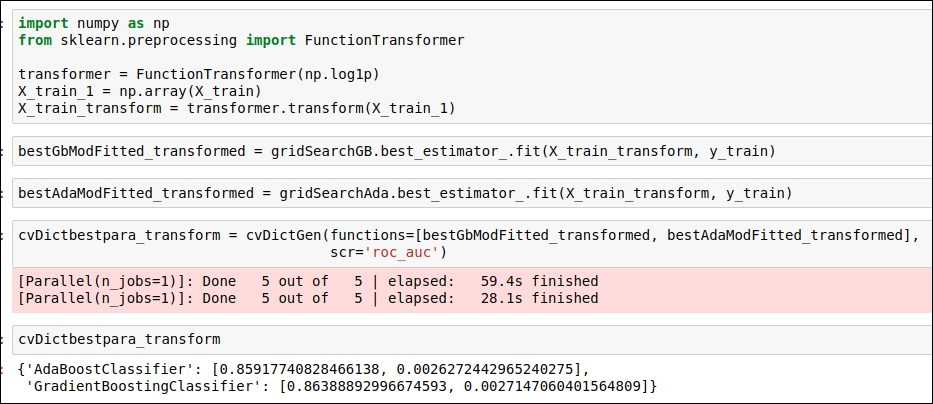Figure 1.63: Code snippet for log(p+1) transformation of features.

I have also tested the ROC-AUC accuracy on the validation dataset, which gives us a minor change in accuracy.

#### Voting-based ensemble ML model

In this section, we will use a voting-based ensemble classifier. The scikit-learn library already has a module available for this. So, we implement a voting-based ML model for both untransformed features as well as transformed features. Let's see which version scores better on the validation dataset. You can refer to the code snippet given in the following figure: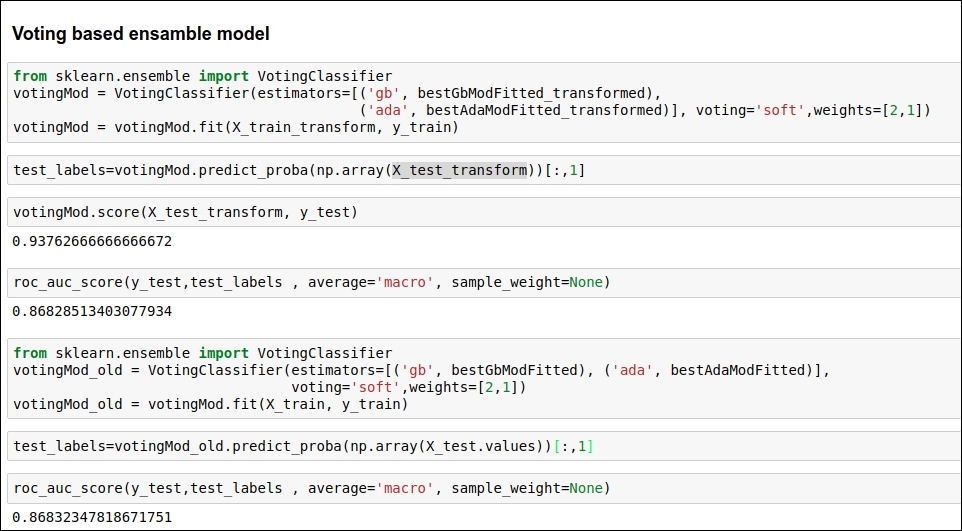Figure 1.64: Code snippet for a voting based ensemble classifier

Here, we are using two parameters: weight 2 for GradientBoosting and 1 for the AdaBoost algorithm. I have also set the voting parameter as soft so classifiers can be more collaborative.

We are almost done with trying out our best approach using a voting mechanism. In the next section, we will run our ML model on a real testing dataset. So let's do some real testing!

#### Running ML models on real test data

Here, we will be testing the accuracy of a voting-based ML model on our testing dataset. In the first iteration, we are not going to take log transformation for the test dataset, and in the second iteration, we are going to take log transformation for the test dataset. In both cases, we will generate the probability for the target class. Here, we are generating probability because we want to know how much of a chance there is of a particular person defaulting on their loan in the next 2 years. We will save the predicted probability in a `csv` file.

You can see the code for performing testing in the following figure: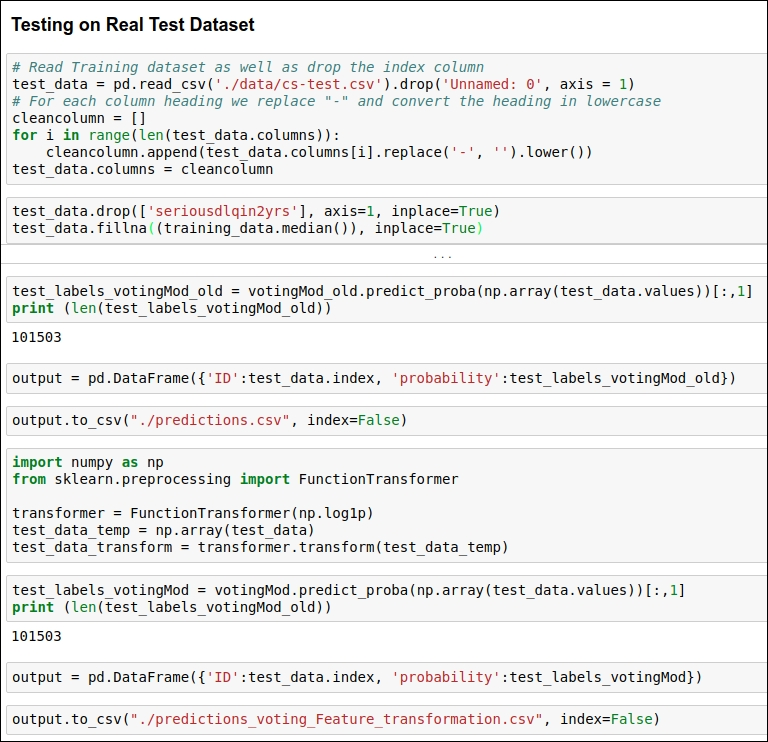Figure 1.65: Code snippet for testing

If you can see Figure 1.64 then you come to know that here, we have achieved 86% accuracy. This score is by far the most efficient accuracy as per industry standards.

## Summary

In this chapter, we looked at how to analyze a dataset using various statistical techniques. After that, we obtained a basic approach and, by using that approach, we developed a model that didn't even achieve the baseline. So, we figured out what had gone wrong in the approach and tried another approach, which solved the issues of our baseline model. Then, we evaluated that approach and optimized the hyper parameters using cross-validation and ensemble techniques in order to achieve the best possible outcome for this application. Finally, we found out the best possible approach, which gave us state-of-the-art results. You can find all of the code for this on GitHub at https://github.com/jalajthanaki/credit-risk-modelling. You can find all the installation related information at https://github.com/jalajthanaki/credit-risk-modelling/blob/master/README.md.

In the next chapter, we will look at another very interesting application of the analytics domain: predicting the stock price of a given share. Doesn't that sound interesting? We will also use some modern machine learning (ML) and deep learning (DL) approaches in order to develop stock price prediction application, so get ready for that as well!